38道 31道 6道

# 第 1 天 栈与队列

## 09. 用两个栈实现队列

### 题目

示例 1：

[[],,[],[]]

[[],[],,,[],[]]



### 解答

• 成员变量：维护两个栈 inStack 和 outStack，其中 inStack 支持队首插入(appendTail)操作，outStack 支持队尾删除(deleteHead)操作
• 构造方法（CQueue）：初始化 inStack 和 outStack 为空
• 队首插入元素（appendTail）：inStack 直接插入元素
• 当 outStack 不为空： outStack 中仍有已完成倒序的元素，因此直接返回 outStack 的栈顶元素。
• 当 outStack 为空，inStack为空： 即两个栈都为空，无元素，因此返回 -1 。
• 当 outStack 为空， inStack不为空，：将 inStack 里的所有元素弹出插入到 outStack 里，实现元素倒序，并返回栈 outStack 的栈顶元素。
class CQueue {
//两个栈，一个出栈，一个入栈
Stack<Integer> inStack;
Stack<Integer> outStack;

public CQueue() {
// 初始化栈
inStack = new Stack<>();
outStack = new Stack<>();
}

public void appendTail(int value) {
inStack.push(value);
}

if(!outStack.isEmpty()){
return outStack.pop();
}
else{
if (inStack.isEmpty()){
return -1;
}
//将inStack的所有值放入outStack
while(!inStack.isEmpty()){
outStack.push(inStack.pop());
}
return outStack.pop();
}
}
}


## 30. 包含min函数的栈

### 题目

示例:
MinStack minStack = new MinStack();
minStack.push(-2);
minStack.push(0);
minStack.push(-3);
minStack.min();   --> 返回 -3.
minStack.pop();
minStack.top();   --> 返回 0.
minStack.min();   --> 返回 -2.


### 解答

class MinStack {
Stack<Integer> dataStack; // 数据栈
Stack<Integer> minStack; // 辅助栈，记录每次有元素进栈后或者出栈后，元素的最小值
public MinStack() {
// 初始化辅助栈和数据栈
dataStack = new Stack<>();
minStack = new Stack<>();
}
public void push(int x) {
dataStack.push(x);// 数据栈，进栈
// 如果辅助栈为空，或者辅助栈栈顶 > x，则将 x 设置为最小值，即进辅助栈
if(minStack.isEmpty() || minStack.peek() > x){
minStack.push(x);
}else{// 如果辅助栈栈顶 < x, 则继续将上次的最小值再入一次辅助栈
minStack.push(minStack.peek());
}
}

public void pop() {
minStack.pop();// 辅助栈，出栈
dataStack.pop();// 数据栈，出栈
}

public int top() {
return dataStack.peek();
}

public int min() {
return minStack.peek();
}
}


# 第 2 天 链表

## 06. 从尾到头打印链表

### 题目

示例 1：



### 解答

/**
* public class ListNode {
*     int val;
*     ListNode next;
*     ListNode(int x) { val = x; }
* }
*/
class Solution {
Stack<ListNode> stack = new Stack<ListNode>();
}
int size = stack.size();
int out[] = new int[size];
for(int i = 0; i < size; i++){
out[i] = stack.pop().val;
}
return out;
}
}


## 24. 反转链表

### 题目

示例:



### 解答

#### 方法1：迭代（双指针）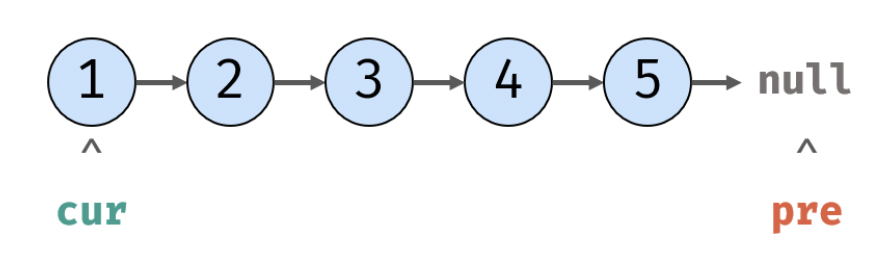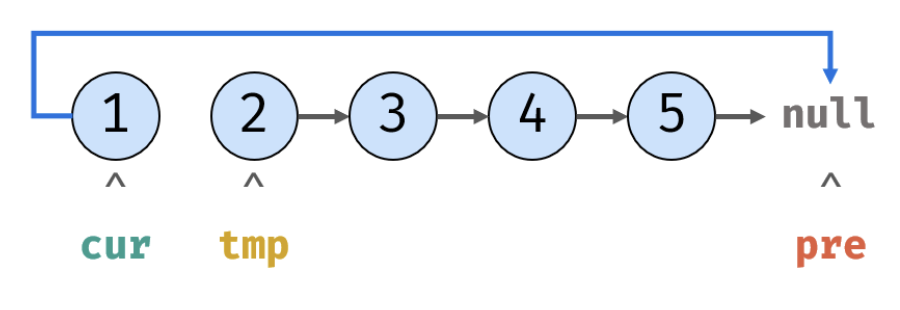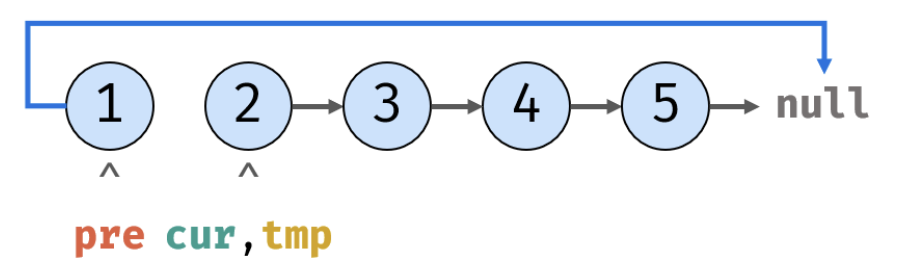class Solution {
ListNode pre = null, cur = head; //初始化pre和cur分别指向null和头节点
while(cur != null) {
ListNode temp = cur.next; // 暂存后继节点 cur.next
cur.next = pre;           // 修改 next 引用指向
pre = cur;                // pre 暂存 cur，即 pre 移动到下一个节点
cur = temp;               // cur 访问下一节点
}
return pre;                   // 返回新的头引用
}
}


#### 方法2：递归

• 终止条件：当前节点和后继为空，则返回尾节点 （即反转链表的头节点）；

• 此后，每次函数在返回的过程中，让当前结点的下一个结点的 next 指针指向当前节点。

• 同时让当前结点的 next 指针指向 NULL ，从而实现从链表尾部开始的局部反转

class Solution {
}
}
}


## 35. 复杂链表的复制

### 题目

输入：head = [[7,null],[13,0],[11,4],[10,2],[1,0]]



输入：head = [[1,1],[2,1]]



输入：head = [[3,null],[3,0],[3,null]]



输入：head = []



### 解答

#### 方法1：哈希表/Map

• **1.**若头结点 head 为空，直接返回 null
• 2.初始化：定义一个哈希表，键为链表的原节点，值为新复制的节点。节点 cur 指向头节点 head
• 3.复制链表：遍历原链表，将复制的节点全部存入哈希表中
• 4.构建新链表的引用指向：再次遍历原链表，构建新链表，确定 next 和 random 指针的指向
• 5.返回值： 新链表的头节点
class Solution {
// 1. 若头结点为空，直接返回 null
// 2. 初始化
Map<Node, Node> map = new HashMap<>();
// 3. 复制各节点，并建立 “原节点 -> 新节点” 的 Map 映射
while(cur != null) {
map.put(cur, new Node(cur.val));
cur = cur.next;
}
// 4. 构建新链表的 next 和 random 指向
while(cur != null) {
map.get(cur).next = map.get(cur.next);
map.get(cur).random = map.get(cur.random);
cur = cur.next;
}
// 5. 返回新链表的头节点
}
}


#### 方法2：拼接 + 拆分

• 1.复制各节点，构建拼接链表:

• 2.构建新链表各节点的 random 指向：

• 3.拆分原 / 新链表：

• 4.返回新链表的头节点 res 即可。
class Solution {
// 1. 复制各节点，并构建拼接链表
while(cur != null) {
Node tmp = new Node(cur.val);
tmp.next = cur.next;
cur.next = tmp;
cur = tmp.next;
}
// 2. 构建各新节点的 random 指向
while(cur != null) {
if(cur.random != null)
cur.next.random = cur.random.next;
cur = cur.next.next;
}
// 3. 拆分两链表
while(cur.next != null) {
pre.next = pre.next.next;
cur.next = cur.next.next;
pre = pre.next;
cur = cur.next;
}
pre.next = null; // 单独处理原链表尾节点
return res;      // 返回新链表头节点
}
}


# 第 3 天 字符串

## 05. 替换空格

### 题目

示例 1：



### 解答

#### 方法1：遍历

class Solution {
public String replaceSpace(String s) {
StringBuilder res = new StringBuilder();
for(int i = 0 ; i < s.length(); i++){
if(s.charAt(i) == ' '){
res.append("%20");
}else{
res.append(s.charAt(i));
}
}
return res.toString();
}
}


#### 方法2：替换

class Solution {
public String replaceSpace(String s) {
return s.replace(" ", "%20");
}
}


## 58 - II. 左旋转字符串

### 题目

示例 1：



### 解答

#### 方法1：切片

class Solution {
public String reverseLeftWords(String s, int n) {
return s.substring(n, s.length()) + s.substring(0, n);
}
}


#### 方法2：遍历

class Solution {
public String reverseLeftWords(String s, int n) {
StringBuilder res = new StringBuilder();
for(int i = n; i< s.length(); i++){
res.append(s.charAt(i));
}
for(int i = 0; i<n; i++){
res.append(s.charAt(i));
}
return res.toString();
}
}


#### 方法3：利用求余运算

class Solution {
public String reverseLeftWords(String s, int n) {
StringBuilder res = new StringBuilder();
for(int i = n; i < n + s.length(); i++)
res.append(s.charAt(i % s.length()));
return res.toString();
}
}


# 第 4 天 查找算法(简单)

## 03. 数组中重复的数字

### 题目

输入：
[2, 3, 1, 0, 2, 5, 3]



### 解答

#### 方法1：哈希表/Set

• 如果添加失败，说明该元素已经在集合中，因此该元素是重复元素，返回该元素
• 反之则没有重复
class Solution {
public int findRepeatNumber(int[] nums) {
Set<Integer> set = new HashSet<>();
for (int num : nums) {
return num;
}
}
return 0;
}
}


#### 方法2：索引匹配

• 由题意可知：长度为 n 的数组 nums 里的所有数字都在 0～n-1 的范围内
• 因此每个元素都可以按照其对应的下标进行存取
• 当其对应的下标被相同的元素占用时，则该元素重复

• 遍历数组 nums ，设索引初始值为 i = 0

• 若 nums[i] = i： 说明此数字已在对应索引位置，无需交换，因此跳过

• 若 nums[nums[i]] = nums[i]： 代表索引 nums[i] 处和索引 i 处的元素值都为 nums[i] ，即找到一组重复值，返回此值 nums[i]

• 否则： 交换索引为 i 和 nums[i] 的元素值，将此数字交换至对应索引位置

• 若遍历完毕尚未返回，则返回 -1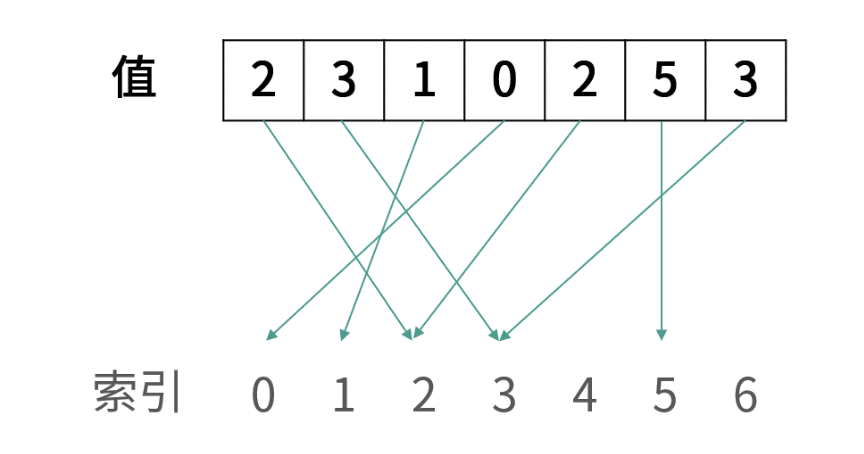class Solution {
public int findRepeatNumber(int[] nums) {
int i = 0;
while(i < nums.length) {
//当前元素正好在其所对应的位置上
if(nums[i] == i) {
i++;
continue;
}
//当前元素所对应的位置的值和当前元素相同，找到重复元素。
if(nums[nums[i]] == nums[i]) return nums[i];
//交换两个元素，重复循环，继续对当前下标的元素进行下标匹配。
int tmp = nums[i];
nums[i] = nums[tmp];
nums[tmp] = tmp;
}
return -1;
}
}


## 53 - I. 在排序数组中查找数字 I

### 题目

输入: nums = [5,7,7,8,8,10], target = 8



输入: nums = [5,7,7,8,8,10], target = 6



### 解答

class Solution {
public int search(int[] nums, int target) {
int count = 0 ;
for(int i = 0; i<nums.length;i++){
if(nums[i] == target){
count++;
}
}
return count;
}
}


## 53 - II. 0～n-1中缺失的数字

### 题目

输入: [0,1,3]



输入: [0,1,2,3,4,5,6,7,9]



### 解答

class Solution {
public int missingNumber(int[] nums) {
for(int i = 0; i < nums.length; i++){
if (i != nums[i])
return i;
}
return  nums.length;
}
}


# 第 5 天 查找算法(中等)

## 04. 二维数组中的查找

### 题目

[
[1,   4,  7, 11, 15],
[2,   5,  8, 12, 19],
[3,   6,  9, 16, 22],
[10, 13, 14, 17, 24],
[18, 21, 23, 26, 30]
]


### 解答

• 从矩阵 matrix 左下角元素（索引设为 (i, j) ）开始遍历，并与目标值对比：
• matrix[i][j] > target 时，则 target 在该行的上方，执行 i--，即消去该行元素；
• matrix[i][j] < target 时，则 target 在该行的右方，执行 j++，即消去该列元素；
• matrix[i][j] = target 时，返回 true，代表找到目标值。
• 若行索引或列索引越界，则代表矩阵中无目标值，返回 false。
class Solution {
public boolean findNumberIn2DArray(int[][] matrix, int target) {
int i = matrix.length - 1, j = 0;
while(i >= 0 && j < matrix.length){
if(matrix[i][j] > target) i--;
else if (matrix[i][j] < target) j++;
else return true;
}
return false;
}
}


### 复杂度

• 时间复杂度： O(M+N)

• M 和 N 分别为矩阵行数和列数，此算法最多循环 M+N 次。
• 空间复杂度： O(1)

• i， j 指针使用常数大小额外空间。

## 11. 旋转数组的最小数字

### 题目

输入：numbers = [3,4,5,1,2]



输入：numbers = [2,2,2,0,1]



### 解答

• 初始化： 声明 low，high 双指针分别指向 numbers 数组左右两端；
• 循环二分： 设 pivot = (low + high) / 2 为每次二分的中点（ “/“ 代表向下取整除法），可分为以下三种情况：
• 当 nums[pivot] > nums[high] 时： 旋转点 x 一定在 [pivot + 1, high] 闭区间内，因此执行 low = pivot + 1；
• 当 nums[pivot] < nums[high] 时： 旋转点 x 一定在 [low, pivot] 闭区间内，因此执行 high = pivot；
• 当 nums[pivot] = nums[high] 时： 无法判断 pivot 在哪个排序数组中，即无法判断旋转点 x 在 [low, pivot] 还是 [pivot + 1, high] 区间中。解决方案： 执行 high = high - 1 缩小判断范围，分析见下文。
• 返回值： 当 low = high 时跳出二分循环，并返回旋转点的值 nums[low] 即可。
class Solution {
public int minArray(int[] numbers) {
int low = 0;
int high = numbers.length - 1;
while (low < high) {
// int pivot = (low + high) / 2;
int pivot = low + (high - low) / 2; //防止low + high造成整型越界
if (numbers[pivot] < numbers[high]) {
high = pivot;
} else if (numbers[pivot] > numbers[high]) {
low = pivot + 1;
} else {
high -= 1;
}
}
return numbers[low];
}
}


### 复杂度

• 时间复杂度：O(logn)

• 空间复杂度：O(1)

## 50. 第一个只出现一次的字符

### 题目

输入：s = "abaccdeff"



输入：s = ""



### 解答

#### 方法1：哈希表/Map

1. 遍历字符串 s ，使用哈希表统计各字符出现次数。
2. 再遍历字符串 s ，在哈希表中找到首个 “数量为 1 的字符”，并返回。
class Solution {
public char firstUniqChar(String s) {
HashMap<Character,Integer> map = new HashMap<>();
char[] S = s.toCharArray();
for(char i : S)
map.put(i,map.getOrDefault(i,0)+1);
for (char i : S)
if(map.get(i)==1)
return i;
return ' ';
}
}


#### 方法2：有序哈希表

class Solution {
public char firstUniqChar(String s) {
Map<Character, Integer> map = new LinkedHashMap<>();
char[] S = s.toCharArray();
for(char i : S)
map.put(i,map.getOrDefault(i,0)+1);
for(Map.Entry<Character, Integer> d : map.entrySet()){
if(d.getValue()==1)
return d.getKey();
}
return ' ';
}
}


### 复杂度

• 时间复杂度：O(n)，其中 n 是字符串 s 的长度。

• 空间复杂度：O(1)， s 只包含小写字母，因此最多有 26 个不同字符，HashMap 存储需占用 O(26) = O(1) 的额外空间。

# 第6天 搜索与回溯算法（简单）

## 32 - I. 从上到下打印二叉树

### 题目

   3
/ \
9  20
/  \
15   7


[3,9,20,15,7]

### 解答

• 特例处理： 当树的根节点为空，则直接返回空列表 [] ；
• 初始化： 结果列表 res = [] ，包含根节点的队列 queue = [root] ；
• BFS 循环： 当队列 queue 为空时跳出；
• 出队： 队首元素出队，记为 node；
• 打印： 将 node.val 添加至列表 tmp 尾部；
• 添加子节点： 若 node 的左（右）子节点不为空，则将左（右）子节点加入队列 queue ；
• 返回值： 返回打印结果列表 res 即可。
/**
* Definition for a binary tree node.
* public class TreeNode {
*     int val;
*     TreeNode left;
*     TreeNode right;
*     TreeNode(int x) { val = x; }
* }
*/
class Solution {
public int[] levelOrder(TreeNode root) {
if(root == null) return new int;// 空树则返回空数组
Queue<TreeNode> queue = new LinkedList<>();// 借助一个队列，通过 BFS 实现按层遍历二叉树
ArrayList<Integer> tmp = new ArrayList<>();// 申请一个动态数组 ArrayList 动态添加节点值
while(!queue.isEmpty()){
TreeNode node = queue.poll();// 取出当前队首元素
}
// 将 ArrayList 转为 int 数组并返回
int[] res = new int[tmp.size()];
for(int i = 0; i < res.length; i++)
res[i] = tmp.get(i);
return res;
}
}


### 复杂度

• 时间复杂度 O(N) ： N 为二叉树的节点数量，即 BFS 需循环 N 次。
• 空间复杂度 O(N)： 最差情况下，即当树为平衡二叉树时，最多有 N/2 个树节点同时在 queue 中，使用 O(N) 大小的额外空间。

## 32 - II. 从上到下打印二叉树 II

### 题目

    3
/ \
9  20
/  \
15   7


[
,
[9,20],
[15,7]
]


### 解答

• 特例处理： 当根节点为空，则返回空列表 [] ；
• 初始化： 打印结果列表 res = [] ，包含根节点的队列 queue = [root] ；
• BFS 循环： 当队列 queue 为空时跳出；
• 新建一个临时列表 tmp ，用于存储当前层打印结果；
• 当前层打印循环： 循环次数为当前层节点数（即队列 queue 长度）；
• 出队： 队首元素出队，记为 node；
• 打印： 将 node.val 添加至 tmp 尾部；
• 添加子节点： 若 node 的左（右）子节点不为空，则将左（右）子节点加入队列 queue ；
• 将当前层结果 tmp 添加入 res 。
• 返回值： 返回打印结果列表 res 即可。
class Solution {
public List<List<Integer>> levelOrder(TreeNode root) {
List<List<Integer>> res = new ArrayList<>();
if(root == null) return res;
while(!queue.isEmpty()) {
List<Integer> tmp = new ArrayList<>();
for(int i = queue.size(); i > 0; i--) {
TreeNode node = queue.poll();
}
}
return res;
}
}


### 复杂度

• 时间复杂度 O(N)： N 为二叉树的节点数量，即 BFS 需循环 N 次。
• 空间复杂度 O(N) ： 最差情况下，即当树为平衡二叉树时，最多有 N/2 个树节点同时在 queue 中，使用 O(N) 大小的额外空间。

## 32 - III. 从上到下打印二叉树 III

### 题目

    3
/ \
9  20
/  \
15   7


[
,
[20,9],
[15,7]
]


### 解答

#### 方法1：层序遍历 + 双端队列

• 奇数层 则添加至 tmp 尾部 ，
• 偶数层 则添加至 tmp 头部 。

• 特例处理： 当树的根节点为空，则直接返回空列表 [] ；
• 初始化： 打印结果空列表 res ，包含根节点的队列 queue ；
• BFS 循环： 当 queue 为空时跳出；
• 新建双端队列 tmp ，用于临时存储当前层打印结果；
• 当前层打印循环： 循环次数为当前层节点数（即 queue 长度）；
• 出队： 队首元素出队，记为 node；
• 打印： 若为奇数层，将 node.val 添加至 tmp 尾部；否则，添加至 tmp 头部
• 添加子节点： 若 node 的左（右）子节点不为空，则加入 queue ；
• 将当前层结果 tmp 添加入 res 。
• 返回值： 返回打印结果列表 res 即可；
/**
* Definition for a binary tree node.
* public class TreeNode {
*     int val;
*     TreeNode left;
*     TreeNode right;
*     TreeNode(int x) { val = x; }
* }
*/
class Solution {
public List<List<Integer>> levelOrder(TreeNode root) {
List<List<Integer>> res = new ArrayList<>();
if(root == null) return res;
while(!queue.isEmpty()) {
for(int i = queue.size(); i > 0; i--) {
TreeNode node = queue.poll();
if(res.size() % 2 == 0) tmp.addLast(node.val); // 奇数层，添加元素至队列尾部
}
}
return res;
}
}


#### 方法2：层序遍历 + 倒序

class Solution {
public List<List<Integer>> levelOrder(TreeNode root) {
List<List<Integer>> res = new ArrayList<>();
while(!queue.isEmpty()) {
List<Integer> tmp = new ArrayList<>();
for(int i = queue.size(); i > 0; i--) {
TreeNode node = queue.poll();
}
if(res.size() % 2 == 1) Collections.reverse(tmp);
}
return res;
}
}


### 复杂度

#### 方法1

• 时间复杂度 O(N)： N 为二叉树的节点数量，即 BFS 需循环 N 次，占用 O(N)；

双端队列的队首和队尾的添加和删除操作的时间复杂度均为 O(1)。

• 空间复杂度 O(N) ： 最差情况下，即当树为满二叉树时，最多有N/2 个树节点 同时 在 queue 中，使用 O(N) 大小的额外空间。

#### 方法2

• 时间复杂度 O(N)： N 为二叉树的节点数量，即 BFS 需循环 N 次，占用 O(N)。

共完成少于 N 个节点的倒序操作，占用 O(N)。

• 空间复杂度 O(N) ： 最差情况下，即当树为满二叉树时，最多有 N/2 个树节点同时在 queue 中，使用 O(N) 大小的额外空间。

# 第 7 天 搜索与回溯算法（简单）

## 26.树的子结构

### 题目

B是A的子结构， 即 A中有出现和B相同的结构和节点值。

    3
/ \
4   5
/ \
1   2


  4
/
1


输入：A = [1,2,3], B = [3,1]



输入：A = [3,4,5,1,2], B = [4,1]



### 解答

recur(A, B) 函数：

1. 终止条件：
1. 当节点 B 为空：说明树 B 已匹配完成（越过叶子节点），因此返回 true ；
2. 当节点 A 为空：说明已经越过树 A 叶子节点，即匹配失败，返回 false ；
3. 当节点 A 和 B 的值不同：说明匹配失败，返回 false ；
2. 返回值：
1. 判断 A和 B 的子节点是否相等，即 recur(A.left, B.left)
2. 判断 A 和 B 的子节点是否相等，即 recur(A.right, B.right)

isSubStructure(A, B) 函数：

1. 特例处理： 当 树 A 为空 树 B 为空 时，直接返回 false ；
2. 返回值： 若树 B 是树 A 的子结构，则必满足以下三种情况之一，因此用或 || 连接；
1. 节点 A 为根节点的子树 包含树 B ，对应 recur(A, B)
2. 树 B 是 树 A 左子树 的子结构，对应 isSubStructure(A.left, B)
3. 树 B 是 树 A 右子树 的子结构，对应 isSubStructure(A.right, B)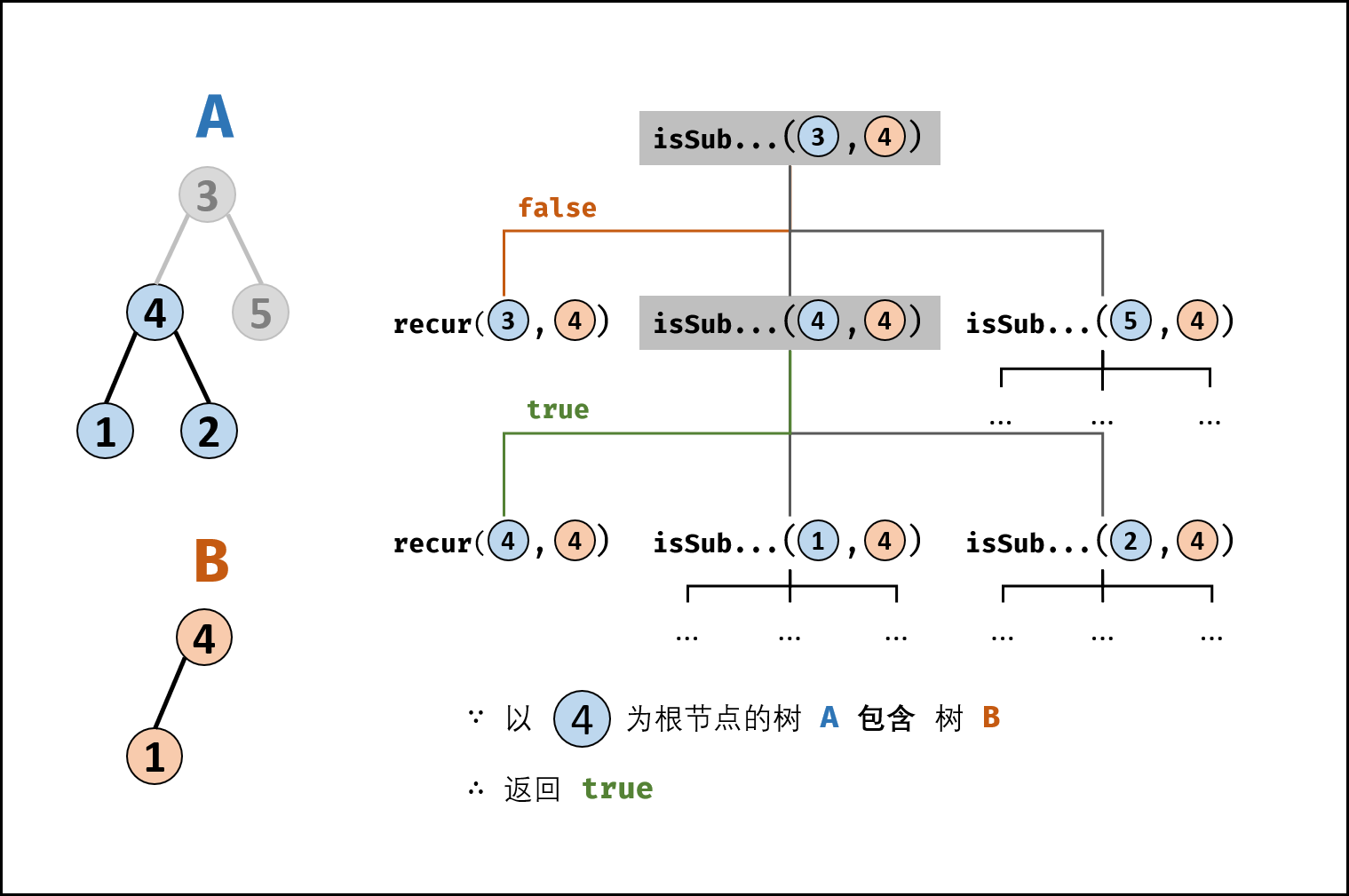/**
* Definition for a binary tree node.
* public class TreeNode {
*     int val;
*     TreeNode left;
*     TreeNode right;
*     TreeNode(int x) { val = x; }
* }
*/
class Solution {
public boolean isSubStructure(TreeNode A, TreeNode B) {
// 若A与B其中一个为空,立即返回false
if(A == null || B == null) {
return false;
}
// B为A的子结构有3种情况,满足任意一种即可:
// 1.B的子结构起点为A的根节点,此时结果为recur(A,B)
// 2.B的子结构起点隐藏在A的左子树中,而不是直接为A的根节点,此时结果为isSubStructure(A.left, B)
// 3.B的子结构起点隐藏在A的右子树中,此时结果为isSubStructure(A.right, B)
return recur(A, B) || isSubStructure(A.left, B) || isSubStructure(A.right, B);
}

/*
判断B是否为A的子结构,其中B子结构的起点为A的根节点
*/
private boolean recur(TreeNode A, TreeNode B) {
// 若B走完了,说明查找完毕,B为A的子结构
if(B == null) {
return true;
}
// 若B不为空并且A为空或者A与B的值不相等,直接可以判断B不是A的子结构
if(A == null || A.val != B.val) {
return false;
}
// 当A与B当前节点值相等,若要判断B为A的子结构
// 还需要判断B的左子树是否为A左子树的子结构 && B的右子树是否为A右子树的子结构
// 若两者都满足就说明B是A的子结构,并且该子结构以A根节点为起点
return recur(A.left, B.left) && recur(A.right, B.right);
}
}


### 复杂度

• 时间复杂度 O(MN)： 其中 M,N 分别为树 A 和 树 B 的节点数量；先序遍历树 A 占用 O(M) ，每次调用 recur(A, B) 判断占用 O(N)。
• 空间复杂度 O(M)： 当树 A 和树 B 都退化为链表时，递归调用深度最大。当 M ≤ N 时，遍历树 A 与递归判断的总递归深度为 M ；当 M > N 时，最差情况为遍历至树 A 叶子节点，此时总递归深度为 M。

## 27.二叉树的镜像

### 题目

    4
/   \
2     7
/ \   / \


1 3 6 9

    4
/   \
7     2
/ \   / \


9 6 3 1

输入：root = [4,2,7,1,3,6,9]



### 解答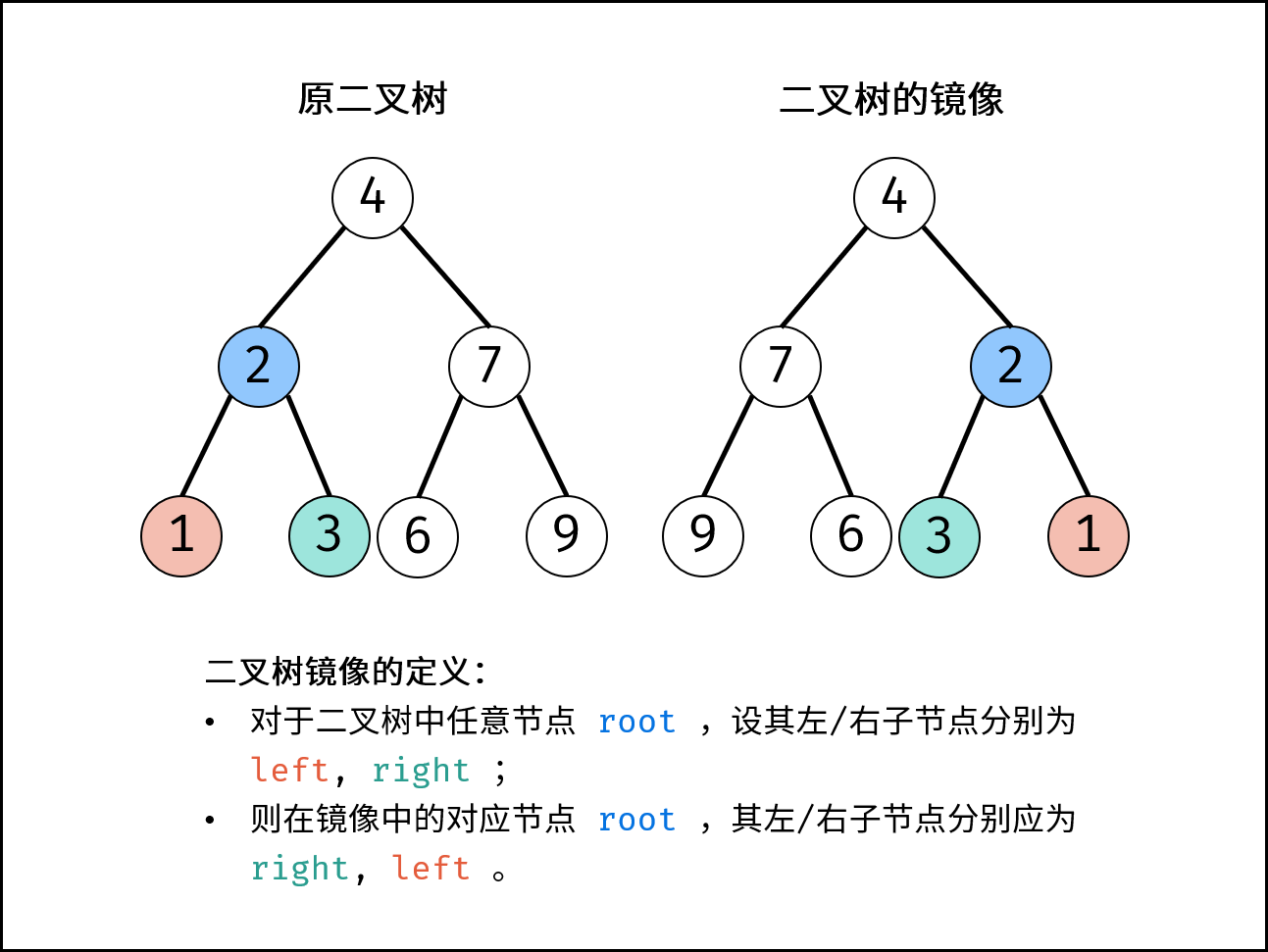#### 方法1：递归法

/**
* Definition for a binary tree node.
* public class TreeNode {
*     int val;
*     TreeNode left;
*     TreeNode right;
*     TreeNode(int x) { val = x; }
* }
*/
class Solution {
public TreeNode mirrorTree(TreeNode root) {
if(root == null) return null;
//从上到下直接交换左右子树
TreeNode tmp = root.left;
root.left = root.right;
root.right = tmp;

mirrorTree(root.left);
mirrorTree(root.right);
return root;
}
}


#### 方法2：辅助栈

• 特例处理： 当 root 为空时，直接返回 null；
• 初始化： 栈，并加入根节点 root。
• 循环交换： 当栈 stack 为空时跳出；
• 出栈： 记为 node ；
• 添加子节点： 将 node 左和右子节点入栈；
• 交换： 交换 node 的左 / 右子节点。
• 返回值： 返回根节点 root 。
class Solution {
public TreeNode mirrorTree(TreeNode root) {
if(root == null) return null;
Stack<TreeNode> stack = new Stack<>();
while(!stack.isEmpty()) {
TreeNode node = stack.pop();
TreeNode tmp = node.left;
node.left = node.right;
node.right = tmp;
}
return root;
}
}


### 复杂度

#### 方法1

• 时间复杂度 O(N)： 其中 N 为二叉树的节点数量，建立二叉树镜像需要遍历树的所有节点，占用 O(N) 时间。
• 空间复杂度 O(N)： 最差情况下（当二叉树退化为链表），递归时系统需使用 O(N) 大小的栈空间。

#### 方法2

• 时间复杂度 O(N) ： 其中 N 为二叉树的节点数量，建立二叉树镜像需要遍历树的所有节点，占用 O(N) 时间。
• 空间复杂度 O(N) ： 最差情况下，栈 stack 最多同时存储 N+1/2个节点，占用 O(N) 额外空间。

## 28.对称的二叉树

### 题目

   1
/ \
2   2
/ \ / \


3 4 4 3

   1
/ \
2   2
\   \
3    3


输入：root = [1,2,2,3,4,4,3]



输入：root = [1,2,2,null,3,null,3]



### 解答

• L.val = R.val： 即此两对称节点值相等。
• L.left.val = R.right.val：即 L 的左子节点和 R 的右子节点对称；
• L.right.val = R.left.val ：即 L 的右子节点和 R 的左子节点对称。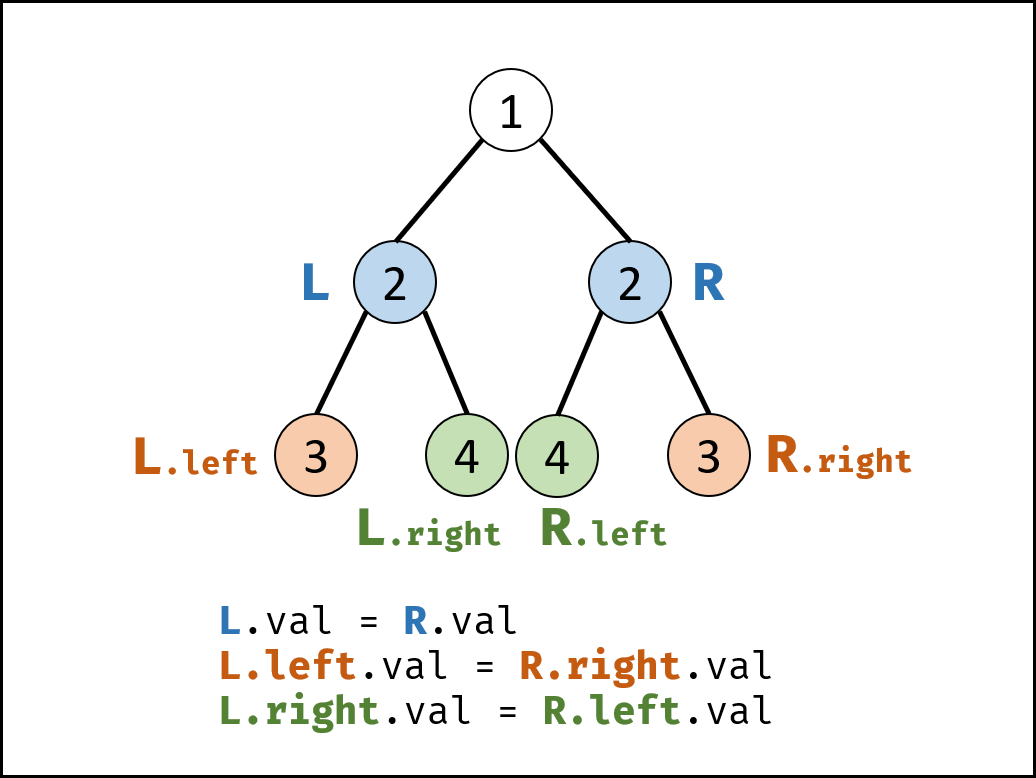isSymmetric(root)

• 特例处理： 若根节点 root 为空，则直接返回 true 。
• 返回值： 即 isSame(root.left, root.right) ;

isSame(L, R)

• 终止条件：
• 当 L 和 R 同时越过叶节点： 此树从顶至底的节点都对称，因此返回 true ；
• 当 L 或 R 中只有一个越过叶节点： 此树不对称，因此返回 false ；
• 当节点 L 值 不等于节点 R 值： 此树不对称，因此返回 false ；
• 递推工作：
• 判断两节点 L.left 和 R.right 是否对称，即 isSame(L.left, R.right)
• 判断两节点 L.right 和 R.left 是否对称，即 isSame(L.right, R.left)
• 返回值： 两对节点都对称时，才是对称树，因此用与逻辑符 && 连接。
class Solution {
public boolean isSymmetric(TreeNode root) {
return root == null ? true : isSame(root.left, root.right);
}
boolean isSame(TreeNode L, TreeNode R) {
if(L == null && R == null) return true;
if(L == null || R == null || L.val != R.val) return false;
return isSame(L.left, R.right) && isSame(L.right, R.left);
}
}


### 复杂度

• 时间复杂度 O(N) ： 其中 N 为二叉树的节点数量，每次执行 isSame() 可以判断一对节点是否对称，因此最多调用 N/2 次 isSame() 方法。
• 空间复杂度 O(N)： 最差情况下，二叉树退化为链表，系统使用 O(N) 大小的栈空间。

# 第 8 天 动态规划（简单）

## 10- I. 斐波那契数列

### 题目

F(0) = 0,   F(1) = 1
F(N) = F(N - 1) + F(N - 2), 其中 N > 1.


输入：n = 2



输入：n = 5



### 解答

#### 方法1：动态规划

class Solution {
public int fib(int n) {
if(n == 0 || n==1) return n;
int[] dp = new int[n + 1];
dp = 0;
dp = 1;
for(int i = 2; i <= n; i++){
dp[i] = dp[i-1] + dp[i-2];
dp[i] %= 1000000007;
}
return dp[n];
}
}

//优化
class Solution {
public int fib(int n) {
int fn0 = 0, fn1 = 1, sum;
for(int i = 0; i <= n; i++){
sum = (fn0 + fn1) % 1000000007;
fn0 = fn1;
fn1 = sum;
}
return fn1;
}
}


。。。

### 复杂度

#### 方法1

• 时间复杂度 O(N) ： 计算 f(n) 需循环 n 次，每轮循环内计算操作使用 O(1) 。
• 空间复杂度 O(1) ： 几个标志变量使用常数大小的额外空间。

#### 方法2

• 时间复杂度：O(logn)
• 空间复杂度：O(1)

## 10- II. 青蛙跳台阶问题

### 题目

输入：n = 2



输入：n = 7



输入：n = 0



### 解答class Solution {
public int numWays(int n) {
if(n == 0 || n==1) return 1;
int[] dp = new int[n + 1];
dp = 1;
dp = 1;
for(int i = 2; i <= n; i++){
dp[i] = dp[i-1] + dp[i-2];
dp[i] %= 1000000007;
}
return dp[n];
}
}

//优化
class Solution {
public int numWays(int n) {
int a = 1, b = 1, sum;
for(int i = 0; i < n; i++){
sum = (a + b) % 1000000007;
a = b;
b = sum;
}
return a;
}
}


### 复杂度

• 时间复杂度 O(N) ： 计算 f(n) 需循环 n 次，每轮循环内计算操作使用 O(1) 。
• 空间复杂度 O(1)： 几个标志变量使用常数大小的额外空间。

## 63.股票的最大利润

### 题目

输入: [7,1,5,3,6,4]



输入: [7,6,4,3,1]



### 解答

#### 方法1：暴力法

public class Solution {
public int maxProfit(int[] prices) {
int maxprofit = 0;
for (int i = 0; i < prices.length - 1; i++) {
for (int j = i + 1; j < prices.length; j++) {
int profit = prices[j] - prices[i];
if (profit > maxprofit) {
maxprofit = profit;
}
}
}
return maxprofit;
}
}


#### 方法2：动态规划dp[i] = max⁡(dp[i−1], prices[i] − min⁡(prices[0:i]))

class Solution {
public int maxProfit(int[] prices) {
if(prices.length == 0)return 0;
int[] dp = new int[prices.length];
dp = 0;
int min = prices;
for(int i = 1; i < prices.length; i++){
min = Math.min(min,prices[i]);
dp[i] = Math.max(dp[i-1],prices[i]-min);
}
return dp[prices.length-1];
}
}


• 时间复杂度降低： 前 iii 日的最低价格 min⁡(prices[0:i]) 时间复杂度为 O(i)。而在遍历 prices 时，可以借助一个变量（记为成本 cost ）每日更新最低价格。优化后的转移方程为：

dp[i] = max⁡(dp[i−1],prices[i] - min⁡(cost,prices[i])

• 空间复杂度降低： 由于 dp[i] 只与 dp[i−1]， prices[i], cost 相关，因此可使用一个变量（记为利润 profit）代替 dp 列表。优化后的转移方程为：

profit = max⁡(profit,prices[i] − min⁡(cost,prices[i])

class Solution {
public int maxProfit(int[] prices) {
int cost = Integer.MAX_VALUE, profit = 0;
for(int price : prices) {
cost = Math.min(cost, price);
profit = Math.max(profit, price - cost);
}
return profit;
}
}


### 复杂度

#### 方法1

• 时间复杂度：O(n2)。循环运行 (n−1)+(n−2)+⋯+2+1=n(n−1)/2 次。
• 空间复杂度：O(1)。只使用了常数个变量。

#### 方法2

• 时间复杂度：O(n)，只需要遍历一次。
• 空间复杂度：O(1)，只使用了常数个变量。

# 第 9 天 动态规划（中等）

## 42.连续子数组的最大和

### 题目

输入: nums = [-2,1,-3,4,-1,2,1,-5,4]



### 解答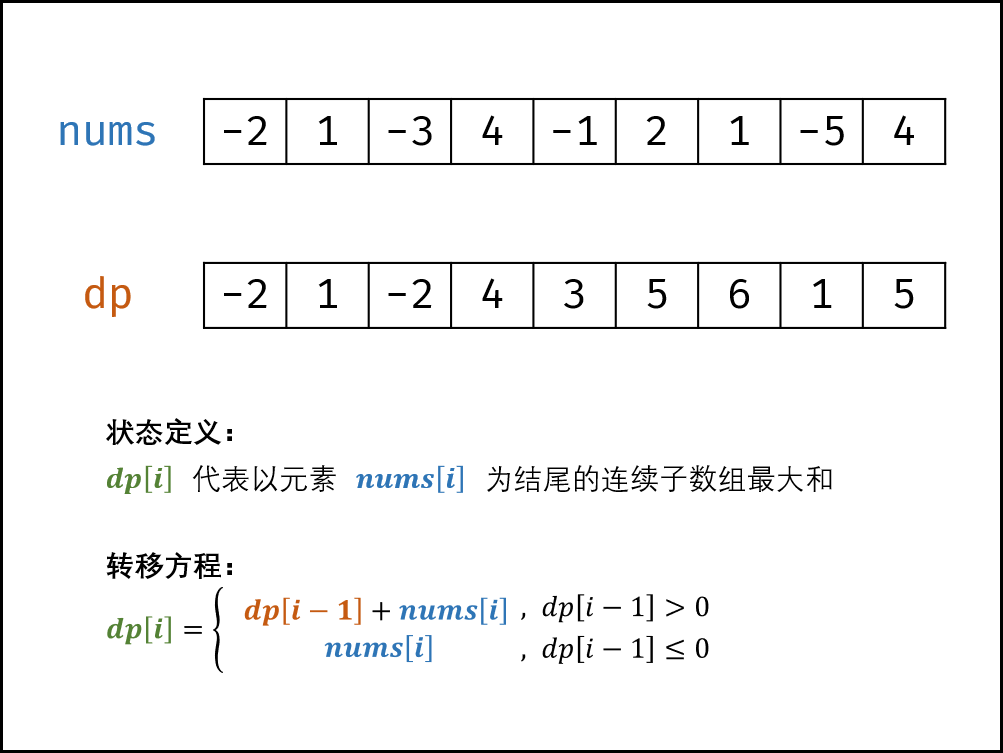class Solution {
public int maxSubArray(int[] nums) {
int dp[] = new int[nums.length];
dp = nums;
int res = dp;
for(int i = 1; i < nums.length; i++){
dp[i] = Math.max(dp[i - 1] + nums[i], nums[i]);
res = Math.max(res, dp[i]);
}
return res;
}
}

//由于 dp[i] 只与 dp[i-1] 和 nums[i] 有关系，因此可以将原数组 nums 用作 dp 列表，即直接在 nums 上修改即可。
class Solution {
public int maxSubArray(int[] nums) {
int res = nums;
for(int i = 1; i < nums.length; i++) {
nums[i] += Math.max(nums[i - 1], 0);
res = Math.max(res, nums[i]);
}
return res;
}
}


### 复杂度

• 时间复杂度 O(N) ： 线性遍历数组 nums 即可获得结果，使用 O(N) 时间。
• 空间复杂度 O(1) ： 使用常数大小的额外空间。

## 47.礼物的最大价值

### 题目

输入:
[
[1,3,1],
[1,5,1],
[4,2,1]
]



### 解答

• 当 i=0 且 j=0 时，为起始元素；
• 当 i=0 且 j≠0 时，为矩阵第一行元素，只可从左边到达；
• 当 i≠0 且 j=0 时，为矩阵第一列元素，只可从上边到达；
• 当 i≠0 且 j≠0 时，可从左边或上边到达；class Solution {
public int maxValue(int[][] grid) {
int m = grid.length;
int n = grid.length;
int [][]dp = new int[m][n];
for(int i = 0; i < m; i++){
for(int j = 0; j < n; j++){
if(i == 0 && j == 0)
dp[i][j] = grid;
else if(i == 0)
dp[i][j] = dp[i][j - 1] + grid[i][j];
else if(j == 0)
dp[i][j] = dp[i - 1][j] + grid[i][j];
else
dp[i][j] = Math.max(dp[i - 1][j], dp[i][j - 1]) + grid[i][j];
}
}
return dp[m - 1][n - 1];
}
}

//优化
/**
由于 dp[i][j] 只与 dp[i-1][j] , dp[i][j-1] , grid[i][j]有关系，因此可以将原矩阵 grid 用作 dp 矩阵，即直接在 grid 上修改即可。应用此方法可省去 dp 矩阵使用的额外空间，因此空间复杂度从 O(MN) 降至 O(1)
**/
class Solution {
public int maxValue(int[][] grid) {
int m = grid.length, n = grid.length;
for(int i = 0; i < m; i++) {
for(int j = 0; j < n; j++) {
if(i == 0 && j == 0) continue;
if(i == 0) grid[i][j] += grid[i][j - 1];
else if(j == 0) grid[i][j] += grid[i - 1][j];
else grid[i][j] += Math.max(grid[i][j - 1], grid[i - 1][j]);
}
}
return grid[m - 1][n - 1];
}
}
//再优化
/**
以上代码逻辑清晰，和转移方程直接对应，但仍可提升效率：当 grid 矩阵很大时， i = 0 或 j = 0 的情况仅占极少数，相当循环每轮都冗余了一次判断。因此，可先初始化矩阵第一行和第一列，再开始遍历递推。
**/
class Solution {
public int maxValue(int[][] grid) {
int m = grid.length, n = grid.length;
for(int j = 1; j < n; j++) // 初始化第一行
grid[j] += grid[j - 1];
for(int i = 1; i < m; i++) // 初始化第一列
grid[i] += grid[i - 1];
for(int i = 1; i < m; i++)
for(int j = 1; j < n; j++)
grid[i][j] += Math.max(grid[i][j - 1], grid[i - 1][j]);
return grid[m - 1][n - 1];
}
}


### 复杂度

• 时间复杂度 O(MN) ： M,N 分别为矩阵行高、列宽；动态规划需遍历整个 grid 矩阵，使用 O(MN) 时间。
• 空间复杂度 O(1) ： 原地修改使用常数大小的额外空间。

# 第 10 天 动态规划（中等）

## 46. 把数字翻译成字符串

### 题目

输入: 12258



### 解答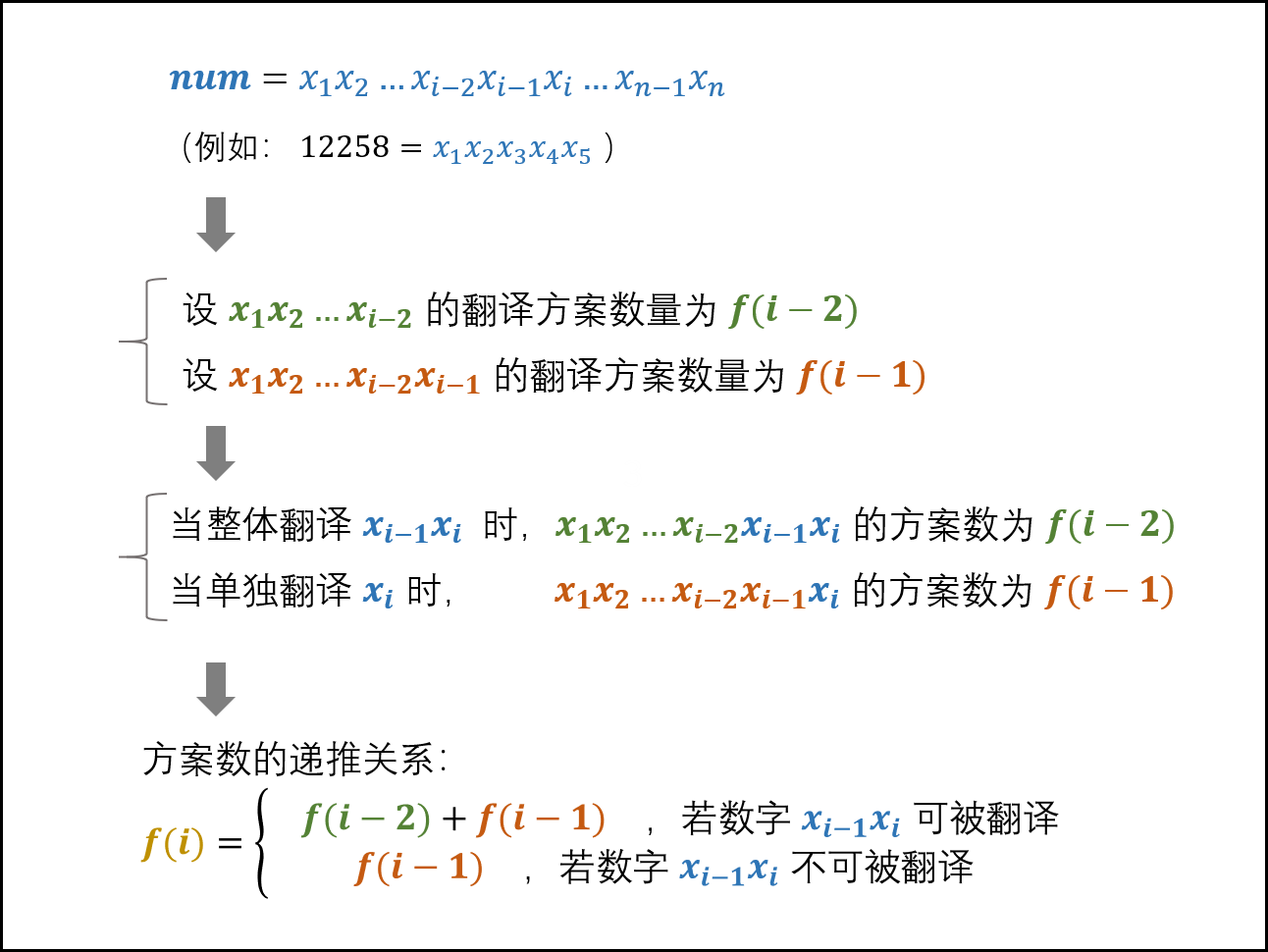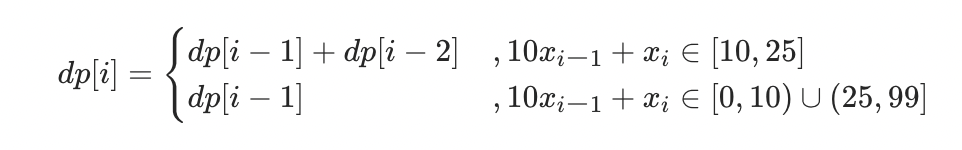Q： 无数字情况 dp = 1 从何而来？
A： 当 num 第 1, 2 位的组成的数字 ∈[10,25] 时，显然应有 2 种翻译方法，即 dp = dp + dp = 2 ，而显然 dp = 1 ，因此推出 dp = 1 。

#### 方法1：字符串遍历

class Solution {
public int translateNum(int num) {
String s = String.valueOf(num);
int []dp = new int[s.length()+1];
dp = dp = 1;
for(int i = 2; i <= s.length(); i++){
String tmp = s.substring(i - 2, i);
if(tmp.compareTo("10") >= 0 && tmp.compareTo("25") <= 0)
dp[i] = dp[i-1] + dp[i-2];
else
dp[i] = dp[i-1];
}
return dp[s.length()];
}
}

//优化
//由于 dp[i]只与 dp[i - 1]和 dp[i - 2]有关，因此可使用变量 a,b,c 分别记录，两变量交替前进即可。此方法可省去 dp 列表使用的 O(N) 的额外空间。
class Solution {
public int translateNum(int num) {
String s = String.valueOf(num);
int a = 1, b = 1;
for(int i = 2; i <= s.length(); i++) {
String tmp = s.substring(i - 2, i);
int c = tmp.compareTo("10") >= 0 && tmp.compareTo("25") <= 0 ? a + b : a;
b = a;
a = c;
}
return a;
}
}


#### 方法2：数字求余




### 复杂度

#### 方法1

• 时间复杂度 O(N) ： N 为字符串 s 的长度（即数字 num 的位数 log⁡(num) ），其决定了循环次数。

• 空间复杂度 O(N)： 字符串 s 和 dp 分别使用 O(N) 大小的额外空间。

#### 方法2

• 时间复杂度 O(N) ： 同方法1
• 空间复杂度 O(1) ： 几个变量使用常数大小的额外空间。

## 48. 最长不含重复字符的子字符

### 解答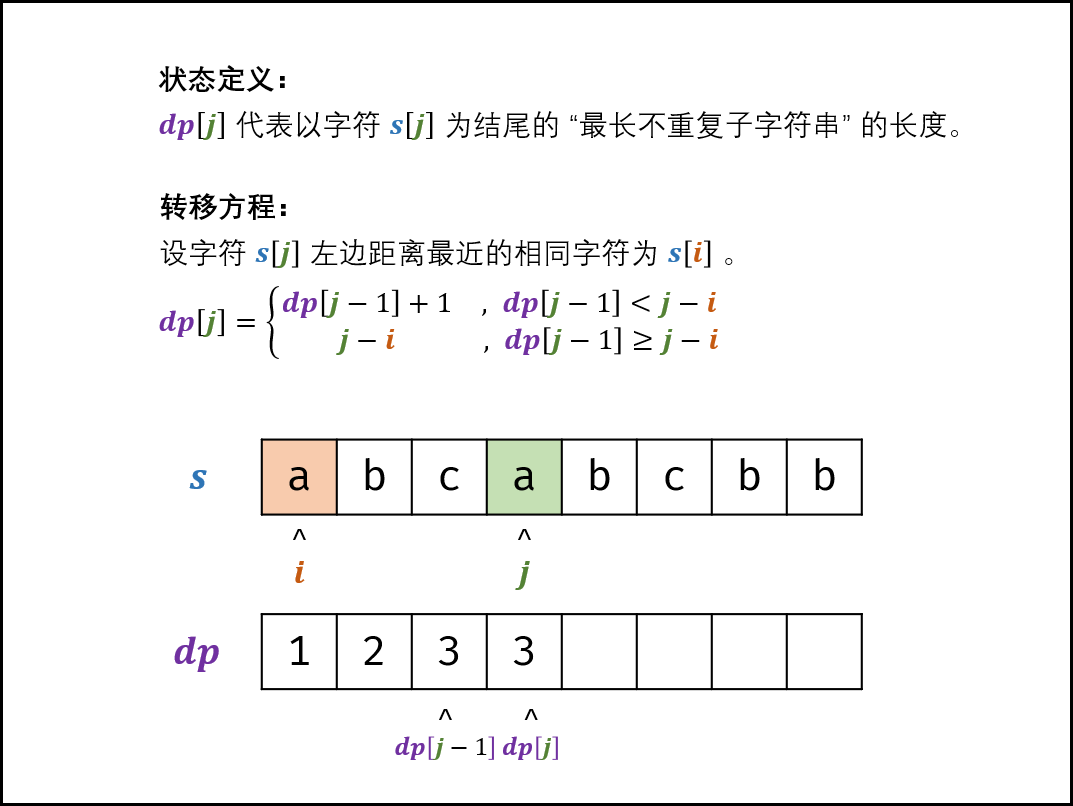#### 方法1：动态规划 + 哈希表

• 哈希表统计： 遍历字符串 s 时，使用哈希表（记为 dic ）统计各字符最后一次出现的索引位置
• 左边界 i 获取方式： 遍历到 s[j] 时，可通过访问哈希表 dic[s[j]] 获取最近的相同字符的索引 i
class Solution {
public int lengthOfLongestSubstring(String s) {
Map<Character,Integer> dic = new HashMap<>();
int tmp = 0, res = 0;
for(int j = 0; j < s.length(); j++){
int i = dic.getOrDefault(s.charAt(j), -1);// 获取索引 i
dic.put(s.charAt(j),j);// 更新哈希表
if(tmp < j - i) tmp = tmp + 1;
else tmp = j - i;
res = Math.max(tmp,res);// max(dp[j - 1], dp[j])
}
return res;
}
}


Java 的 getOrDefault(key, default)， 代表当哈希表包含键 key 时返回对应 value ，不包含时返回默认值 default

# 第 11 天 双指针（简单）

## 18. 删除链表的节点

### 题目

输入: head = [4,5,1,9], val = 5



输入: head = [4,5,1,9], val = 1



### 解答##### 算法流程：
1. 特例处理： 当应删除头节点 head 时，直接返回 head.next 即可。
2. 初始化： pre = head , cur = head.next
3. 定位节点：cur 为空 cur 节点值等于 val 时跳出。
1. 保存当前节点索引，即 pre = cur
2. 遍历下一节点，即 cur = cur.next
4. 删除节点：cur 指向待删除节点，则执行 pre.next = cur.next ；若 cur 指向 null，代表链表中不包含值为 val 的节点。
5. 返回值： 返回链表头部节点 head 即可。
/**
* public class ListNode {
*     int val;
*     ListNode next;
*     ListNode(int x) { val = x; }
* }
*/
class Solution {
public ListNode deleteNode(ListNode head, int val) {
while(cur != null && cur.val != val){
pre = cur;
cur = cur.next;
}
if(cur.val == val)
pre.next = cur.next;
}
}


### 复杂度

• 时间复杂度 O(N)： N 为链表长度，删除操作平均需循环 N/2 次，最差 N 次。
• 空间复杂度 O(1) ： cur, pre 占用常数大小额外空间。

## 22. 链表中倒数第k个节点

### 题目

给定一个链表: 1->2->3->4->5, 和 k = 2.



### 解答

• 先遍历统计链表长度，记为 n ；
• 设置一个指针走 (n-k)步，即可找到链表倒数第 k 个节点。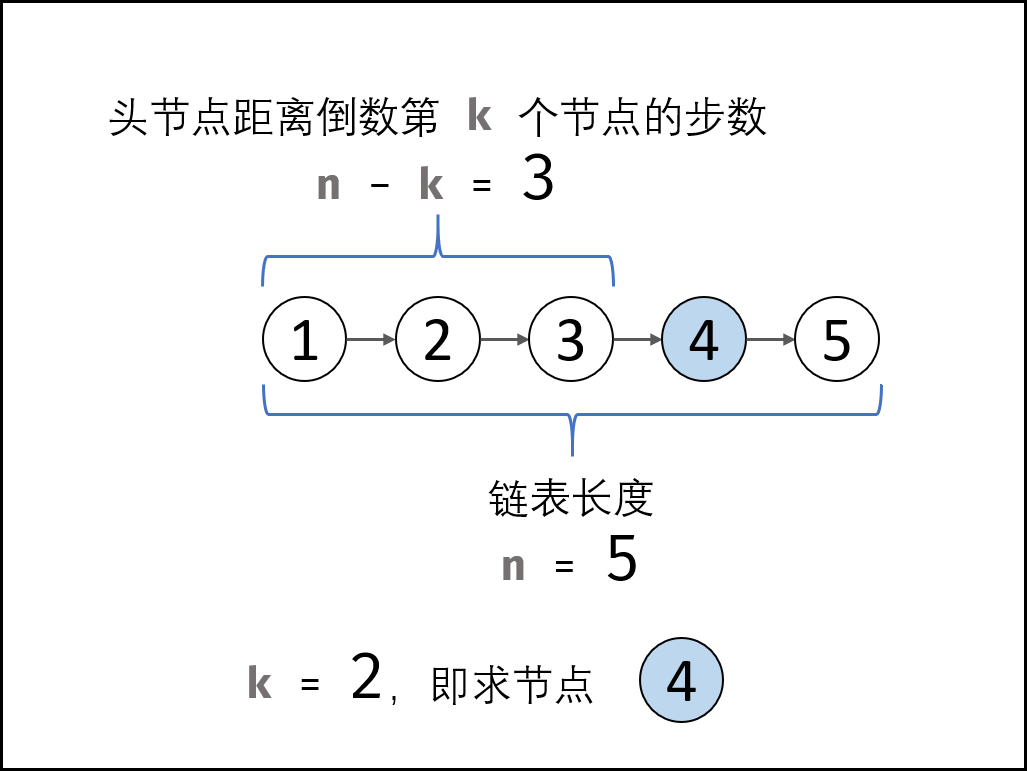##### 算法流程：
1. 初始化： 前指针 former 、后指针 latter ，双指针都指向头节点 head
2. 构建双指针距离： 前指针 former 先向前走 k 步（结束后，双指针 formerlatter 间相距 k 步）。
3. 双指针共同移动： 循环中，双指针 formerlatter 每轮都向前走一步，直至 former 走过链表 尾节点 时跳出（跳出后， latter 与尾节点距离为 k−1，即 latter 指向倒数第 k 个节点）。
4. 返回值： 返回 latter 即可。
/**
* public class ListNode {
*     int val;
*     ListNode next;
*     ListNode(int x) { val = x; }
* }
*/
class Solution {
public ListNode getKthFromEnd(ListNode head, int k) {
for(int i = 0; i < k; i++){
former = former.next;
}
while(former != null){
former = former.next;
latter = latter.next;
}
return latter;
}
}


### 复杂度

• 时间复杂度 O(N) ： N 为链表长度；总体看， former 走了 N 步， latter 走了 (N−k) 步。
• 空间复杂度 O(1) ： 双指针 former , latter 使用常数大小的额外空间。

# 第 12 天 双指针（简单）

## 25. 合并两个排序的链表

### 题目

输入：1->2->4, 1->3->4



### 解答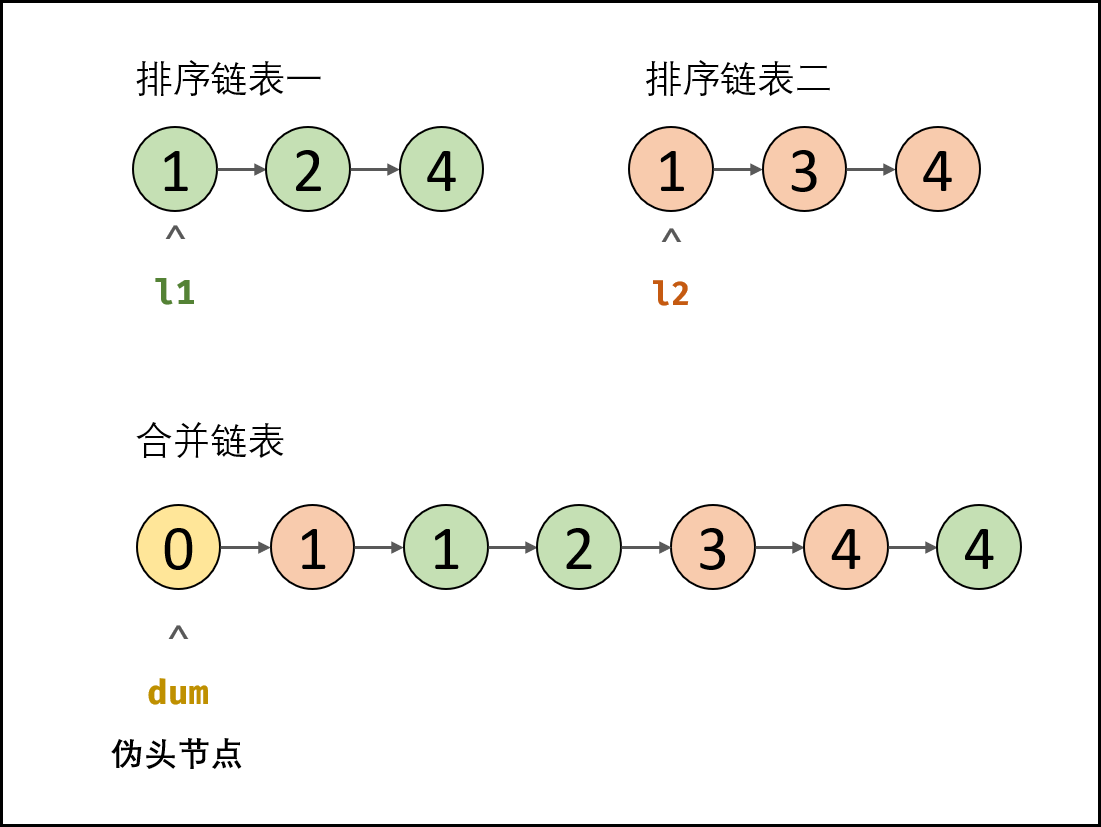##### 算法流程：
1. 初始化： 伪头节点 dum ，节点 cur 指向 dum 。
2. 循环合并： 当 l1 或 l2 为空时跳出；
1. 当 l1.val < l2.val 时： cur 的后继节点指定为 l1，并且 l1 向前走一步；
2. 当 l1.val ≥ l2.val 时： cur 的后继节点指定为 l2，并且 l2 向前走一步 ；
3. 节点 cur 向前走一步，即 cur = cur.next。
3. 合并剩余尾部： 跳出时有两种情况，即 l1 为空 l2 为空。
1. 若 l1 ≠ null ： 将 l1 添加至节点 cur 之后；
2. 否则： 将 l2 添加至节点 cur 之后。
4. 返回值： 合并链表在伪头节点 dum 之后，因此返回 dum.next 即可。
/**
* public class ListNode {
*     int val;
*     ListNode next;
*     ListNode(int x) { val = x; }
* }
*/
class Solution {
public ListNode mergeTwoLists(ListNode l1, ListNode l2) {
ListNode dum = new ListNode(0), cur = dum;
while(l1 != null && l2 != null){
if(l1.val < l2.val) {
cur.next = l1;
l1 = l1.next;
}
else{
cur.next = l2;
l2 = l2.next;
}
cur = cur.next;
}
if(l1 != null) cur.next = l1;
if(l2 != null) cur.next = l2;
return dum.next;
}
}


### 复杂度

• 时间复杂度 O(M+N)： M,N 分别为链表 l1,l2 的长度，合并操作需遍历两链表。
• 空间复杂度 O(1) ： 节点引用 dum , cur 使用常数大小的额外空间。

## 52. 两个链表的第一个公共节点

### 题目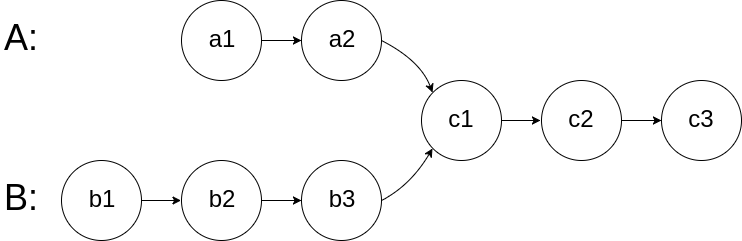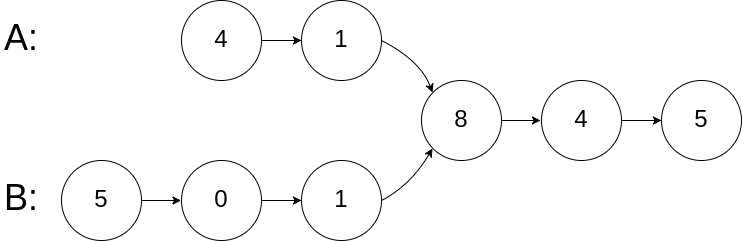输入：intersectVal = 8, listA = [4,1,8,4,5], listB = [5,0,1,8,4,5], skipA = 2, skipB = 3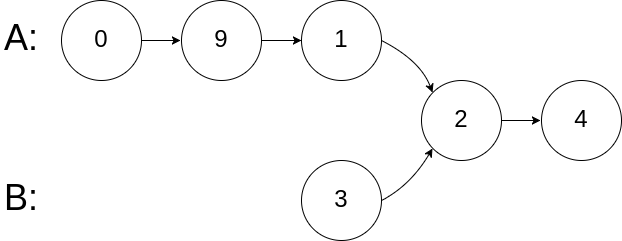输入：intersectVal = 2, listA = [0,9,1,2,4], listB = [3,2,4], skipA = 3, skipB = 1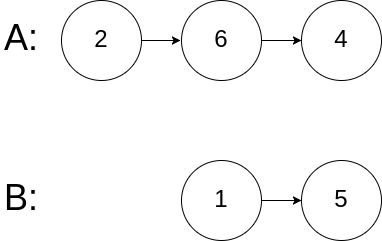输入：intersectVal = 0, listA = [2,6,4], listB = [1,5], skipA = 3, skipB = 2



### 解答

• 头节点 headAnode 前，共有 a−c 个节点；
• 头节点 headBnode 前，共有 b−c 个节点；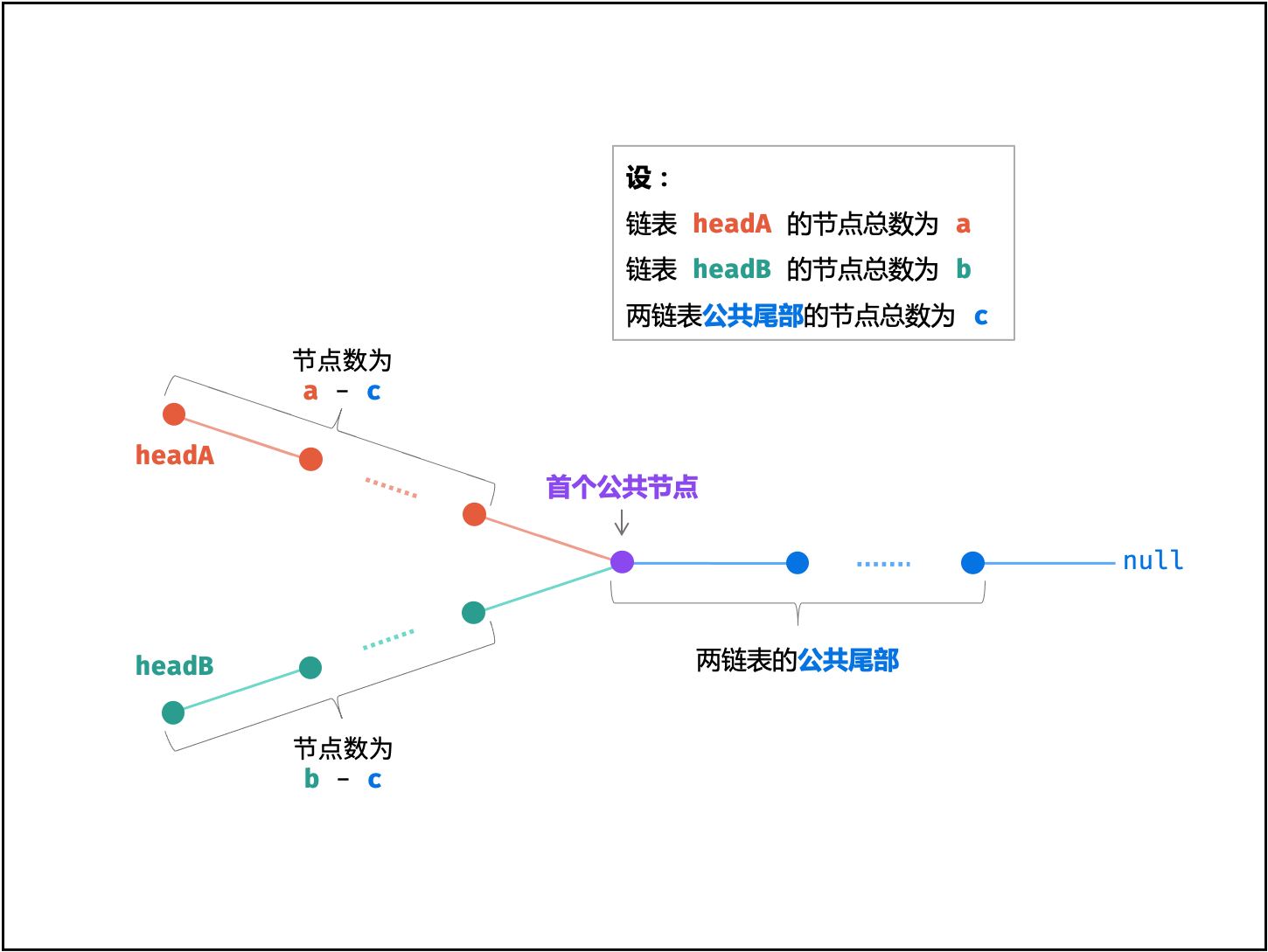• 指针 A 先遍历完链表 headA ，再开始遍历链表 headB ，当走到 node 时，共走步数为：a+(b−c)

• 指针 B 先遍历完链表 headB ，再开始遍历链表 headA ，当走到 node 时，共走步数为：b+(a−c)

a+(b−c) = b+(a−c)，此时指针 A , B 重合 ，并有两种情况：

1. 若两链表 公共尾部 (即 c>0 ) ：指针 A , B 同时指向「第一个公共节点」node
2. 若两链表 公共尾部 (即 c=0) ：指针 A , B 同时指向 null 。

/**
* public class ListNode {
*     int val;
*     ListNode next;
*     ListNode(int x) {
*         val = x;
*         next = null;
*     }
* }
*/
class Solution {
while(A != B){
if(A != null) A = A.next;
if(B != null) B = B.next;
}
return A;
}
}

//简化代码
class Solution {
while(A != B){
A = A != null ? A.next : headB;
B = B != null ? B.next : headA;
}
return A;
}
}


### 复杂度

• 时间复杂度 O(a+b)： 最差情况下（即 ∣a−b∣=1 , c=0 ），此时需遍历 a+b 个节点。
• 空间复杂度 O(1)： 节点指针 A , B 使用常数大小的额外空间。

# 第 13 天 双指针（简单）

## 21. 调整数组顺序使奇数位于偶

### 题目

输入：nums = [1,2,3,4]



### 解答##### 算法流程：
1. 初始化： i , j 双指针，分别指向数组 nums 左右两端；
2. 循环交换： 当 i = j 时跳出；
1. 指针 i 遇到奇数则执行 i = i+1 跳过，直到找到偶数；
2. 指针 j 遇到偶数则执行 j = j−1 跳过，直到找到奇数；
3. 交换 nums[i] 和 nums[j] 值；
3. 返回值： 返回已修改的 nums 数组。

“**1**”的二进制 表示形式为 00000001

• 结果为 1 ，则为奇数
• 结果为 0 ，则为偶数
class Solution {
public int[] exchange(int[] nums) {
int i = 0, j = nums.length - 1, tmp;
while(i < j){
if(nums[i] % 2 != 0){
i++;
}
if(nums[j] % 2 == 0){
j--;
}
if(i < j){
tmp = nums[i];
nums[i] = nums[j];
nums[j] = tmp;
}
}
return nums;
}
}

//优化
class Solution {
public int[] exchange(int[] nums) {
int i = 0, j = nums.length - 1, tmp;
while(i < j) {
while(i < j && (nums[i] & 1) == 1) i++;
while(i < j && (nums[j] & 1) == 0) j--;
tmp = nums[i];
nums[i] = nums[j];
nums[j] = tmp;
}
return nums;
}
}


### 复杂度

• 时间复杂度 O(N) ： NNN 为数组 nums 长度，双指针 i, j 共同遍历整个数组。
• 空间复杂度 O(1) ： 双指针 i, j 使用常数大小的额外空间。

## 57. 和为s的两个数字

### 题目

输入：nums = [2,7,11,15], target = 9



输入：nums = [10,26,30,31,47,60], target = 40



### 解答

##### 算法流程：
1. 初始化： 双指针 i , j 分别指向数组 nums 的左右两端 （俗称对撞双指针）。
2. 循环搜索： 当双指针相遇时跳出；
1. 计算和 sum = nums[i]+nums[j]；
2. 若 sum > target，则指针 j 向左移动，即执行 j=j−1 ；
3. 若 sum < target ，则指针 i 向右移动，即执行 i=i+1 ；
4. 若 sum = target ，立即返回数组 [nums[i],nums[j]] ；
3. 返回空数组，代表无和为 target 的数字组合。
class Solution {
public int[] twoSum(int[] nums, int target) {
int i = 0, j = nums.length -1;
while(i < j){
int sum = nums[i] + nums[j];
if(sum > target) j--;
else if(sum < target) i++;
else return new int[]{nums[i],nums[j]};
}
return null;
//return new int;
}
}


### 复杂度

• 时间复杂度 O(N) ： N 为数组 nums 的长度；双指针共同线性遍历整个数组。
• 空间复杂度 O(1) ： 变量 i, j 使用常数大小的额外空间。

## 58 - I. 翻转单词顺序

### 题目

输入: "the sky is blue"



输入: "  hello world!  "



输入: "a good   example"



### 解答

#### 方法1：调用API

1. 使用 split 将字符串按空格分割成字符串数组；
2. 使用 reverse 将字符串数组进行反转；
3. 使用 join 方法将字符串数组拼成一个字符串。
class Solution {
public String reverseWords(String s) {
// 除去开头和末尾的空白字符
s = s.trim();
// 正则匹配连续的空白字符作为分隔符分割
List<String> wordList = Arrays.asList(s.split("\\s+"));
Collections.reverse(wordList);
return String.join(" ", wordList);
}
}

• Arrays.asList 将数组转化成List集合
• Collections.reverse 反转列表元素
• String.join 用给定的定界符连接数组或列表中所有元素
• \s+”则表示匹配任意多个空白字符。另因为反斜杠在Java里是转义字符，所以在Java里，我们要这么用“\\s+

#### 方法2：双指针

##### 算法解析：
• 倒序遍历字符串 s ，记录单词左右索引边界 i , j ；
• 每确定一个单词的边界，则将其添加至单词列表 res ；
• 最终，将单词列表拼接为字符串，并返回即可。
class Solution {
public String reverseWords(String s) {
s = s.trim(); // 删除首尾空格
int j = s.length() - 1, i = j;
StringBuilder res = new StringBuilder();
while(i >= 0) {
while(i >= 0 && s.charAt(i) != ' ') i--; // 搜索首个空格
res.append(s.substring(i + 1, j + 1) + " "); // 添加单词
while(i >= 0 && s.charAt(i) == ' ') i--; // 跳过单词间空格
j = i; // j 指向下个单词的尾字符
}
return res.toString().trim(); // 转化为字符串，删除尾部空格，并返回
}
}


### 复杂度

#### 方法1

• **时间复杂度O(N)**：其中 N 为字符串 s 的长度。

• **空间复杂度O(N)**：用来存储字符串分割之后的结果。

#### 方法2

• 时间复杂度 O(N)： 其中 N 为字符串 s 的长度，线性遍历字符串。
• 空间复杂度 O(N) ： 新建的 StringBuilder(Java) 中的字符串总长度 ≤N，占用 O(N) 大小的额外空间。

# 第 14 天 搜索与回溯算法（中等）

## 12. 矩阵中的路径

### 题目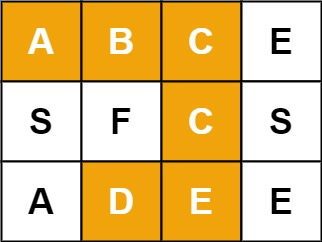输入：board = [["A","B","C","E"],["S","F","C","S"],["A","D","E","E"]], word = "ABCCED"



输入：board = [["a","b"],["c","d"]], word = "abcd"



### 解答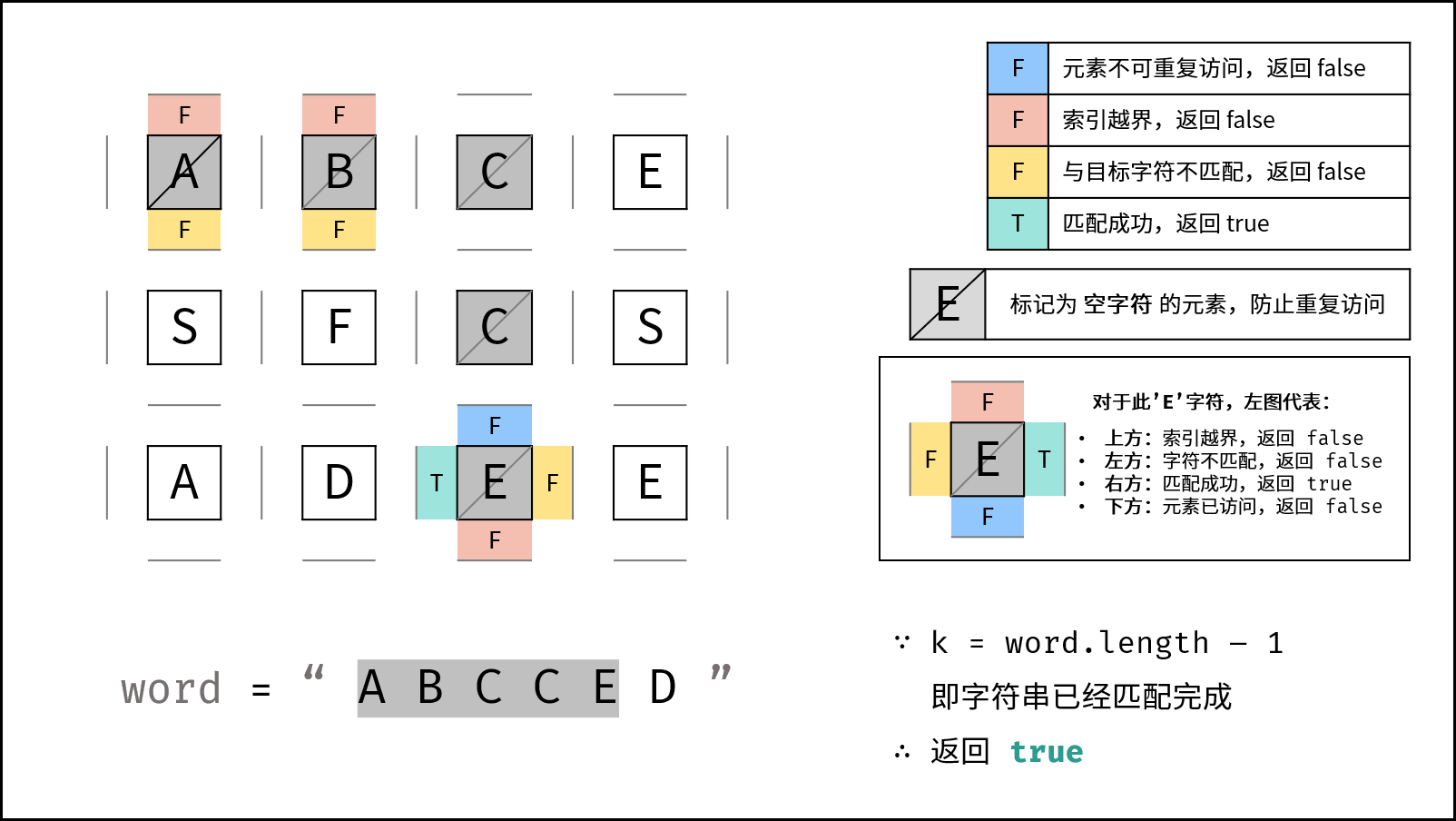##### DFS 解析：
• 递归参数： 当前元素在矩阵 board 中的行列索引 ij ，当前目标字符在 word 中的索引 k
• 终止条件：
1. 返回 false ： (1) 行或列索引越界 (2) 当前矩阵元素与目标字符不同 (3) 当前矩阵元素已访问过 （ (3) 可合并至 (2) ） 。
2. 返回 true ： k = len(word) - 1 ，即字符串 word 已全部匹配。
• 递推工作：
1. 标记当前矩阵元素： 将 board[i][j] 修改为 空字符 '' ，代表此元素已访问过，防止之后搜索时重复访问。
2. 搜索下一单元格： 朝当前元素的 上、下、左、右 四个方向开启下层递归，使用 或 连接 （代表只需找到一条可行路径就直接返回，不再做后续 DFS ），并记录结果至 res
3. 还原当前矩阵元素： 将 board[i][j] 元素还原至初始值，即 word[k]
• 返回值： 返回布尔量 res ，代表是否搜索到目标字符串
class Solution {
public boolean exist(char[][] board, String word) {
char[] words = word.toCharArray();
for(int i = 0; i < board.length; i++) {
for(int j = 0; j < board.length; j++) {
if(dfs(board, words, i, j, 0)) return true;
}
}
return false;
}
boolean dfs(char[][] board, char[] word, int i, int j, int k) {
if(i >= board.length || i < 0 || j >= board.length || j < 0 || board[i][j] != word[k]) return false;
if(k == word.length - 1) return true;
board[i][j] = '\0';
boolean res = dfs(board, word, i + 1, j, k + 1) || dfs(board, word, i - 1, j, k + 1) ||
dfs(board, word, i, j + 1, k + 1) || dfs(board, word, i , j - 1, k + 1);
board[i][j] = word[k];
return res;
}
}


### 复杂度

M,N 分别为矩阵行列大小， K 为字符串 word 长度。

• 时间复杂度 O(3KMN)： 最差情况下，需要遍历矩阵中长度为 K 字符串的所有方案，时间复杂度为 O(3K)；矩阵中共有 MN 个起点，时间复杂度为 O(MN) 。
• 方案数计算： 设字符串长度为 K ，搜索中每个字符有上、下、左、右四个方向可以选择，舍弃回头（上个字符）的方向，剩下 3 种选择，因此方案数的复杂度为 O(3K) 。
• 空间复杂度 O(K) ： 搜索过程中的递归深度不超过 K ，因此系统因函数调用累计使用的栈空间占用 O(K) （因为函数返回后，系统调用的栈空间会释放）。最坏情况下 K=MN，递归深度为 MN ，此时系统栈使用 O(MN) 的额外空间。

## 13. 机器人的运动范围

### 题目

输入：m = 2, n = 3, k = 1



输入：m = 3, n = 1, k = 0



### 解答

class Solution {
public int movingCount(int m, int n, int k) {
// 记录位置是否被遍历过
boolean[][] visited = new boolean[m][n];
return dfs(visited, m, n, 0, 0, k);
}

private int dfs(boolean[][] visited, int m, int n, int i, int j, int k) {
// i >= m || j >= n是边界条件的判断
if (i >= m || j >= n
// visited[i][j]判断这个格子是否被访问过
|| visited[i][j] == true
// 判断当前格子坐标是否满足条件
|| bitSum(i) + bitSum(j) > k) {
return 0;
}
// 标注这个格子被访问过
visited[i][j] = true;
// 沿着当前格子的右边和下边继续访问
//代表从本单元格递归搜索的可达解总数。
return 1 + dfs(visited, m, n, i + 1, j, k) + dfs(visited, m, n, i, j + 1, k);
}
// 计算数位和
private int bitSum(int x) {
int sum = 0;
while(x > 0) {
sum += x % 10;
x /= 10;
}
return sum;
}
}


### 复杂度

• 时间复杂度 O(MN) ： 最差情况下，机器人遍历矩阵所有单元格，此时时间复杂度为 O(MN) 。
• 空间复杂度 O(MN)： 最差情况下， visited 内存储矩阵所有单元格的索引，使用 O(MN) 的额外空间。

# 第 15 天 搜索与回溯算法（中等）

## 34. 二叉树中和为某一值的路径

### 题目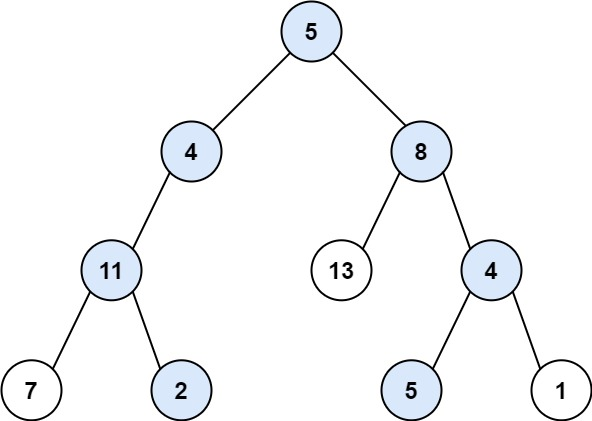输入：root = [5,4,8,11,null,13,4,7,2,null,null,5,1], targetSum = 22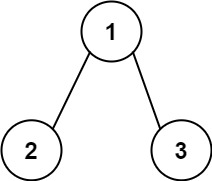输入：root = [1,2,3], targetSum = 5



输入：root = [1,2], targetSum = 0



### 解答

/**
* Definition for a binary tree node.
* public class TreeNode {
*     int val;
*     TreeNode left;
*     TreeNode right;
*     TreeNode() {}
*     TreeNode(int val) { this.val = val; }
*     TreeNode(int val, TreeNode left, TreeNode right) {
*         this.val = val;
*         this.left = left;
*         this.right = right;
*     }
* }
*/
class Solution {
public List<List<Integer>> pathSum(TreeNode root, int target) {
dfs(root, target);
return res;

}
private void dfs(TreeNode root, int target){
if(root == null) return;
target -= root.val;
if(target == 0 && root.left == null && root.right == null)
//因为直接加入，加入的是引用(指向的堆中数据会变化)，需要克隆一份加入
dfs(root.left, target);
dfs(root.right, target);
path.removeLast();// 将本次搜索结果移除，方便其他搜索使用path变量
}
}


### 复杂度

• 时间复杂度 O(N) ： N 为二叉树的节点数，先序遍历需要遍历所有节点。
• 空间复杂度 O(N) ： 最差情况下，即树退化为链表时，path 存储所有树节点，使用 O(N) 额外空间。

## 36. 二叉搜索树与双向链表

### 题目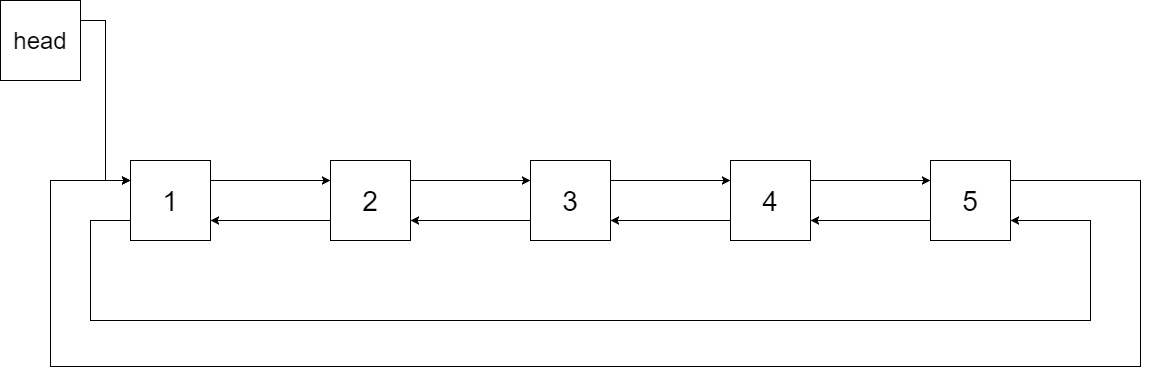### 解答

1. 排序链表： 二叉搜索树的中序遍历为 递增序列
2. 双向链表： 在构建相邻节点的引用关系时，设前驱节点 pre 和当前节点 cur ，不仅应构建 pre.right = cur ，也应构建 cur.left = pre
3. 循环链表： 设链表头节点 head 和尾节点 tail ，则应构建 head.left = tailtail.right = head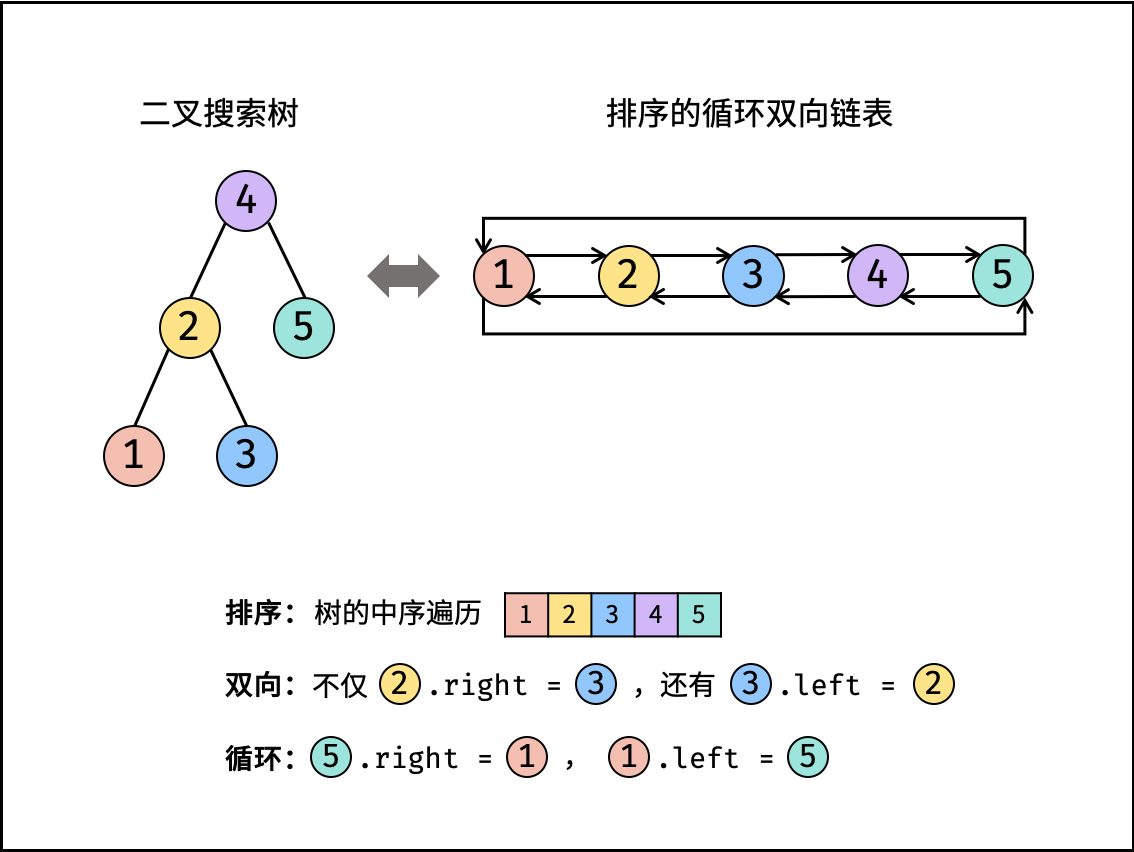class Solution {
public Node treeToDoublyList(Node root) {
if(root == null) return null;
dfs(root);
}
void dfs(Node cur){
if(cur == null) return;
dfs(cur.left);
if(pre != null)
pre.right = cur;
else
cur.left = pre;
pre = cur;
dfs(cur.right);
}
}


### 复杂度

• 时间复杂度 O(N) ： N 为二叉树的节点数，中序遍历需要访问所有节点。
• 空间复杂度 O(N) ： 最差情况下，即树退化为链表时，递归深度达到 N，系统使用 O(N) 栈空间。

## 54.二叉搜索树的第k大节点

### 题目

输入: root = [3,1,4,null,2], k = 1
3
/ \
1   4
\
2



输入: root = [5,3,6,2,4,null,null,1], k = 3
5
/ \
3   6
/ \
2   4
/
1



### 解答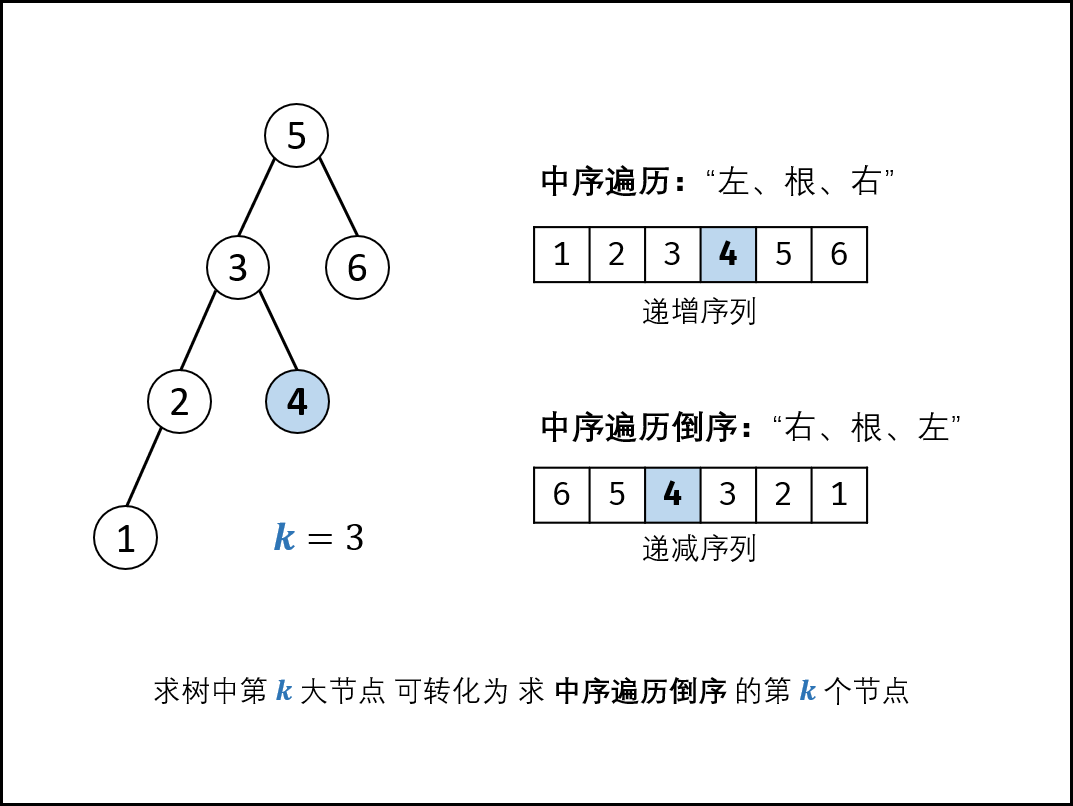class Solution {
int count=0, res=0;//形参k不能随着dfs的迭代而不断变化，为了记录迭代进程和结果，引入类变量count和res。
public int kthLargest(TreeNode root, int k) {
this.count=k;//利用形参值k对类变量count进行初始化
dfs(root);//这里不要引入形参k，dfs中直接使用的是初始值为k的类变量count
return res;
}
public void dfs(TreeNode root){ //中序遍历的倒序
if(root==null||count==0) return;//当root为空或者已经找到了res时，直接返回
dfs(root.right);
if(--count==0){//先--，再判断
res = root.val;
return;//这里的return可以避免之后的无效迭代dfs(root.left);
}
dfs(root.left);
}
}


### 复杂度

• 时间复杂度 O(N)： 当树退化为链表时（全部为右子节点），无论 k 的值大小，递归深度都为 N ，占用 O(N) 时间。
• 空间复杂度 O(N)： 当树退化为链表时（全部为右子节点），系统使用 O(N) 大小的栈空间。

# 第 16 天 排序（简单）

## 45.把数组排成最小的数

### 题目

输入: [10,2]



输入: [3,30,34,5,9]



### 解答

• 若拼接字符串 xy > yx ，则 x 应在 y 右边 ；
• 反之，若 xy < yx，则 x 应在 y 左边 ；
class Solution {
public String minNumber(int[] nums) {
String[] strs = new String[nums.length];
for(int i = 0; i < nums.length; i++){
strs[i] = String.valueOf(nums[i]);
}
Arrays.sort(strs, (x, y) -> (x + y).compareTo(y + x));
StringBuilder res = new StringBuilder();
for(String s : strs){
res.append(s);
}
return res.toString();
}
}


### 复杂度

• 时间复杂度 O(Nlog⁡N) ：N 为最终返回值的字符数量；使用内置函数 Arrays.sort 的平均时间复杂度为 O(Nlog⁡N)，最差为 O(N2)。
• 空间复杂度 O(N) ： 字符串列表 strs 占用线性大小的额外空间。

## 61. 扑克牌中的顺子

### 题目

输入: [1,2,3,4,5]



输入: [0,0,1,2,5]



### 解答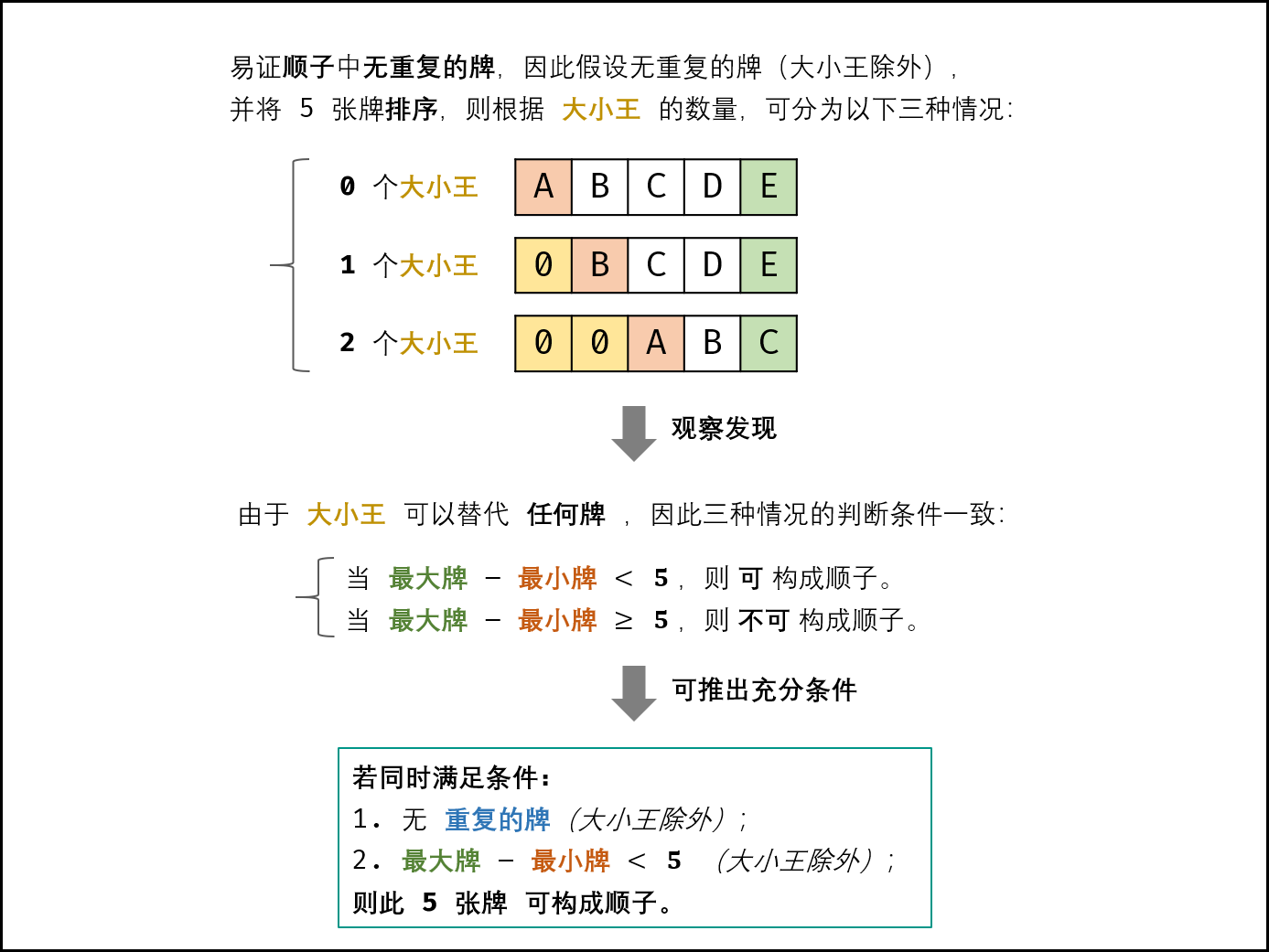• 遍历五张牌，遇到大小王（即 0 ）直接跳过。
• 判别重复： 利用 Set 实现遍历判重， Set 的查找方法的时间复杂度为 O(1) ；
• 获取最大 / 最小的牌： 借助辅助变量 max 和 min ，遍历统计即可。
class Solution {
public boolean isStraight(int[] nums) {
Set<Integer> set = new HashSet<>();
int max = 0, min = 14;
for(int num : nums){
if(num == 0) continue; // 跳过大小王
max = Math.max(max,num);// 最大牌
min = Math.min(min,num);// 最小牌
if(set.contains(num)) return false; // 若有重复，提前返回 false
}
return max - min < 5;// 最大牌 - 最小牌 < 5 则可构成顺子
}
}


### 复杂度

• 时间复杂度 O(N)=O(5)=O(1)： 其中 N 为 nums 长度，本题中 N≡5 ；遍历数组使用 O(N) 时间。
• 空间复杂度 O(N)=O(5)=O(1) ： 用于判重的辅助 Set 使用 O(N) 额外空间。

# 第 17 天 排序（中等）

## 40. 最小的k个数

### 题目

输入：arr = [3,2,1], k = 2



输入：arr = [0,1,2,1], k = 1



### 解答

class Solution {
public int[] getLeastNumbers(int[] arr, int k) {
int[] res = new int[k];
Arrays.sort(arr);
for(int i = 0; i < k; i++){
res[i] = arr[i];
}
return res;
}
}

class Solution {
public int[] getLeastNumbers(int[] arr, int k) {
Arrays.sort(arr);
return Arrays.copyOf(arr, k);
}
}


### 复杂度

• 时间复杂度 O(Nlog⁡N) ：N 为数组长度；使用内置函数 Arrays.sort 的平均时间复杂度为 O(Nlog⁡N)，最差为 O(N2)。
• 空间复杂度 O(N) ： 数组占用线性大小的额外空间。

## 41. 数据流中的中位数

### 题目

[2,3,4] 的中位数是 3

[2,3] 的中位数是 (2 + 3) / 2 = 2.5

• void addNum(int num) - 从数据流中添加一个整数到数据结构中。
• double findMedian() - 返回目前所有元素的中位数。

输入：
[[],,,[],,[]]



输入：
[[],,[],,[]]



### 解答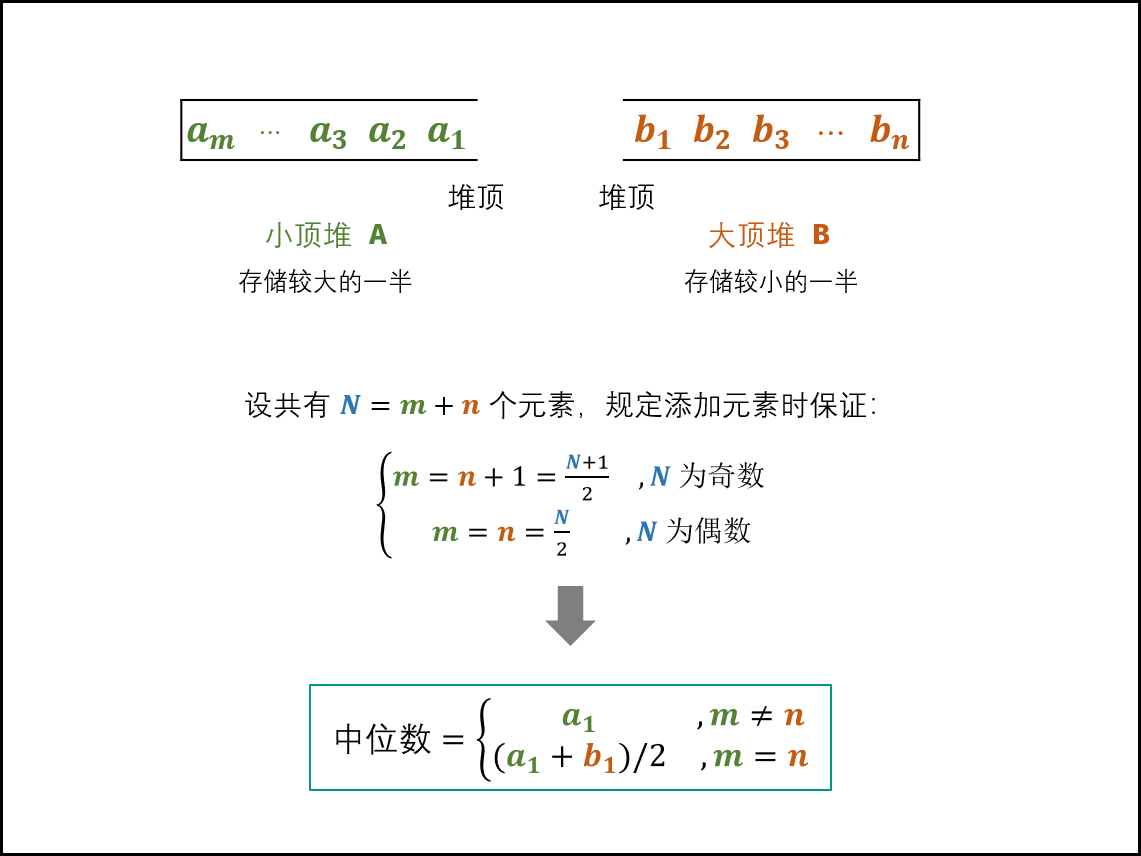class MedianFinder {
Queue<Integer> A, B;
public MedianFinder() {
A = new PriorityQueue<>(); // 小顶堆，保存较大的一半
B = new PriorityQueue<>((x, y) -> (y - x)); // 大顶堆，保存较小的一半
}
if(A.size() != B.size()) {
} else {
}
}
public double findMedian() {
return A.size() != B.size() ? A.peek() : (A.peek() + B.peek()) / 2.0;
}
}


### 复杂度

• 时间复杂度：
• 查找中位数 O(1) ： 获取堆顶元素使用 O(1)时间；
• 添加数字 O(log⁡N)： 堆的插入和弹出操作使用 O(log⁡N) 时间。
• 空间复杂度 O(N) ： 其中 N 为数据流中的元素数量，小顶堆 A 和大顶堆 B 最多同时保存 N 个元素。

# 第 18 天 搜索与回溯算法（中等）

## 55 - I. 二叉树的深度

### 题目

   3
/ \
9  20
/  \
15   7


### 解答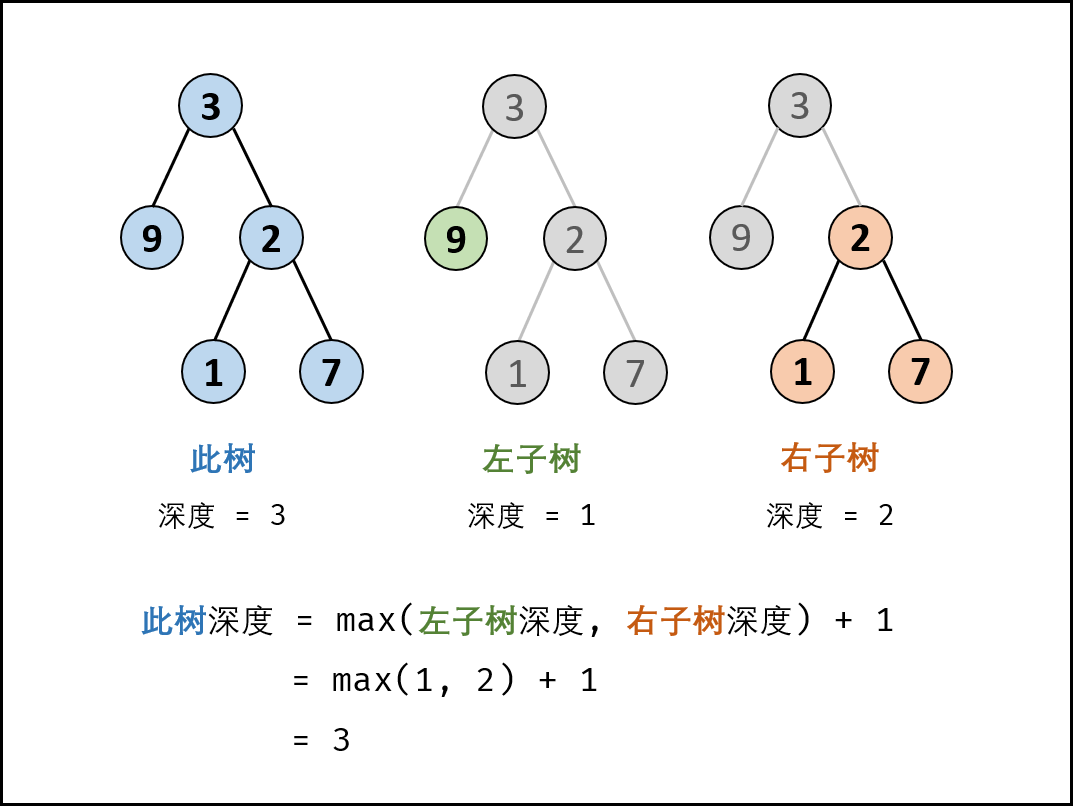class Solution {
public int maxDepth(TreeNode root) {
if(root == null) return 0;
return Math.max(maxDepth(root.left), maxDepth(root.right)) + 1;
}
}


### 复杂度

• 时间复杂度 O(N) ： N 为树的节点数量，计算树的深度需要遍历所有节点。
• 空间复杂度 O(N)： 最差情况下（当树退化为链表时），递归深度可达到 N 。

## 55 - II. 平衡二叉树

### 题目

  3
/ \
9  20
/  \
15   7


     1
/ \
2   2
/ \
3   3
/ \


4 4

### 解答

class Solution {
public boolean isBalanced(TreeNode root) {
if (root == null) return true;
return Math.abs(depth(root.left) - depth(root.right)) <= 1 && isBalanced(root.left) && isBalanced(root.right);
}

private int depth(TreeNode root) {
if (root == null) return 0;
return Math.max(depth(root.left), depth(root.right)) + 1;
}
}


### 复杂度

• 时间复杂度 O(NlogN)：最差情况下（为 “满二叉树” 时），满二叉树高度的复杂度 O(logN)，各层执行 depth(root) 的时间复杂度为 O(N)，总体时间复杂度 == 每层执行复杂度 × 层数复杂度 = O(N×logN) 。

• 空间复杂度 O(N)： 最差情况下（树退化为链表时），系统递归需要使用 O(N) 的栈空间。

# 第 19 天 搜索与回溯算法（中等）

## 64. 求1+2+…+n

### 题目

输入: n = 3



输入: n = 9



### 解答

1+2+…+(n−1)+n 的计算方法主要有三种：平均计算、迭代、递归。

• 平均计算：(1 + n) * n / 2；此计算必须使用 乘除法 ，因此本方法不可取，直接排除。
public int sumNums(int n) {
return (1 + n) * n / 2;
}

• 迭代：循环必须使用 while 或 for，因此本方法不可取，直接排除。
public int sumNums(int n) {
int res = 0;
for(int i = 1; i <= n; i++)
res += i;
return res;
}

• 递归：终止条件需要使用 if ，因此本方法不可取。
public int sumNums(int n) {
if(n == 0) return 0;
n += sumNums(n - 1);
return n;
}

• 正确解答：使用逻辑运算符的短路效应来终止递归

if(A && B)  // 若 A 为 false ，则 B 的判断不会执行（即短路），直接判定 A && B 为 false

if(A || B) // 若 A 为 true ，则 B 的判断不会执行（即短路），直接判定 A || B 为 true


n > 0 && n += sumNums(n - 1) // 当 n = 0 时 n > 0 不成立 ，此时 “短路” ，终止后续递归

class Solution {
public int sumNums(int n) {
boolean flag = n > 0 && (n += sumNums(n - 1)) > 0;
return n;
}
}


### 复杂度

• 时间复杂度 O(n) ： 计算 n+(n−1)+…+2+1 需要开启 n 个递归函数。
• 空间复杂度 O(n) ： 递归深度达到 n ，系统使用 O(n) 大小的额外空间。

## 68 - I. 二叉搜索树的最近公共祖先

### 题目输入: root = [6,2,8,0,4,7,9,null,null,3,5], p = 2, q = 8



输入: root = [6,2,8,0,4,7,9,null,null,3,5], p = 2, q = 4



### 解答

#### 方法1：迭代

• 循环搜索： 当节点 root 为空时跳出；
1. 当 p,q 都在 root 的 右子树 中，则遍历至 root.right ；
2. 否则，当 p,q 都在 root 的 左子树 中，则遍历至 root.left ；
3. 否则，说明找到了 最近公共祖先 ，跳出。
• 返回值： 最近公共祖先 root 。

• 若 root.val<p.val ，则 p 在 root 右子树 中；
• 若 root.val>p.val ，则 p 在 root 左子树 中；
• 若 root.val=p.val ，则 p 和 root 指向 同一节点
class Solution {
public TreeNode lowestCommonAncestor(TreeNode root, TreeNode p, TreeNode q) {
while(root != null) {
if(root.val < p.val && root.val < q.val) // p,q 都在 root 的右子树中
root = root.right; // 遍历至右子节点
else if(root.val > p.val && root.val > q.val) // p,q 都在 root 的左子树中
root = root.left; // 遍历至左子节点
else break;
}
return root;
}
}


#### 方法2：递归

class Solution {
public TreeNode lowestCommonAncestor(TreeNode root, TreeNode p, TreeNode q) {
if(root.val < p.val && root.val < q.val)
return lowestCommonAncestor(root.right, p, q);
if(root.val > p.val && root.val > q.val)
return lowestCommonAncestor(root.left, p, q);
return root;
}
}


### 复杂度

#### 方法1

• 时间复杂度 O(N)： 其中 N 为二叉树节点数；每循环一轮排除一层，二叉搜索树的层数最小为 log⁡N （满二叉树），最大为 N （退化为链表）。
• 空间复杂度 O(1)： 使用常数大小的额外空间。

#### 方法2

• 时间复杂度 O(N)： 其中 N 为二叉树节点数；每循环一轮排除一层，二叉搜索树的层数最小为 log⁡N （满二叉树），最大为 N （退化为链表）。
• 空间复杂度 O(N)： 最差情况下，即树退化为链表时，递归深度达到树的层数 N 。

## 68 - II. 二叉树的最近公共祖先

### 题目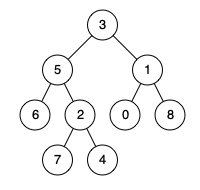输入: root = [3,5,1,6,2,0,8,null,null,7,4], p = 5, q = 1



输入: root = [3,5,1,6,2,0,8,null,null,7,4], p = 5, q = 4



### 解答




# 第 20 天 分治算法（中等）

## 07. 重建二叉树

### 题目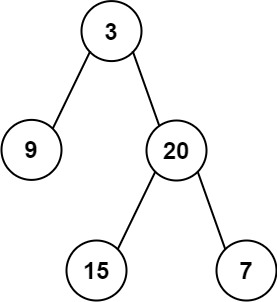Input: preorder = [3,9,20,15,7], inorder = [9,3,15,20,7]
Output: [3,9,20,null,null,15,7]


Input: preorder = [-1], inorder = [-1]
Output: [-1]


### 解答TIPS： pre_root + i - in_left + 1含义为 根节点索引 + 左子树长度 + 1

class Solution {
int[] preorder;//保留的先序遍历，方便递归时依据索引查看先序遍历的值
HashMap<Integer, Integer> dic = new HashMap<>();//存储中序遍历的值与索引
public TreeNode buildTree(int[] preorder, int[] inorder) {
this.preorder = preorder;
//将中序遍历的值及索引放在map中，方便递归时获取左子树与右子树的数量及其根的索引
for(int i = 0; i < inorder.length; i++)
dic.put(inorder[i], i);
return recur(0, 0, inorder.length - 1);//recur(当前根的的索引,子树左边界,子树右边界)
}
TreeNode recur(int pre_root, int in_left, int in_right) {
if(in_left > in_right) return null;                        // 递归终止
TreeNode root = new TreeNode(preorder[pre_root]);          // 建立根节点
int i = dic.get(preorder[pre_root]);                       // 划分根节点、左子树、右子树
//左子树的根的索引为先序中的根节点+1
//左子树的左边界为原来的中序in_left
//左子树的右边界为中序中的根节点索引-1
root.left = recur(pre_root + 1, in_left, i - 1);           // 开启左子树递归
//右子树的根的索引为先序中的当前根位置 + 左子树的长度 + 1
//右子树的左边界为中序中当前根节点+1
//右子树的右边界为中序中原来右子树的边界
root.right = recur(pre_root + i - in_left + 1, i + 1, in_right); // 开启右子树递归
return root;                                           // 回溯返回根节点
}
}


### 复杂度

• 时间复杂度 O(N) ： 其中 N 为树的节点数量。初始化 HashMap 需遍历 inorder ，占用 O(N)。递归共建立 N 个节点，每层递归中的节点建立、搜索操作占用 O(1)，因此使用 O(N)时间。
• 空间复杂度 O(N) ： HashMap 使用 O(N) 额外空间；最差情况下（输入二叉树为链表时），递归深度达到 N ，占用 O(N) 的栈帧空间；因此总共使用 O(N) 空间。

## 16. 数值的整数次方

### 题目

输入：x = 2.00000, n = 10



输入：x = 2.10000, n = 3



输入：x = 2.00000, n = -2



### 解答

• 对于任何十进制正整数 n ，设其二进制为 “bm…b3b2b1
• n = 1b1 + 2b2 + 4b3 + … + 2m−1bm
• xn = x1b1 + 2b2 + 4b3 + … + 2m−1bm = x1b1x2b2x4b3…x2m−1bm

• 计算 x1,x2,x4,…,x2m−1的值： 循环赋值操作 x = x2 即可；
• 获取二进制各位 b1,b2,b3,…,bm 的值： 循环执行以下操作即可。
1. n&1 （与操作）： 判断 n 二进制最右一位是否为 1 ；
2. n>>1 （移位操作）： n 右移一位（可理解为删除最后一位）。

Java 代码中 int32 变量 n∈[−2147483648,2147483647]，因此当 n=−2147483648 时执行 n = −n 会因越界而赋值出错。解决方法是先将 n 存入 long 变量 b ，后面用 b 操作即可。

class Solution {
public double myPow(double x, int n) {
if(x == 0) return 0;
long b = n;
double res = 1.0;
if(b < 0) {
x = 1 / x;
b = -b;
}
while(b > 0) {
if((b & 1) == 1) res *= x;
x *= x;
b >>= 1;
}
return res;
}
}

• 如果 n 为偶数： xn = xn/2 * xn/2* xn%2
• 如果 n 为奇数： xn = xn/2 * xn/2 * xn%2
class Solution {
public double myPow(double x, int n) {
if(n == 0) return 1;
if(n == 1) return x;
if(n == -1) return 1 / x;
double half = myPow(x, n / 2);
double mod = myPow(x, n % 2);
return half * half * mod;
}
}


### 复杂度

• 时间复杂度 O(log2n)： 二分的时间复杂度为对数级别。
• 空间复杂度 O(1) ： res, b 等变量占用常数大小额外空间。

## 33. 二叉搜索树的后序遍历序列

### 题目

    5
/ \
2   6
/ \
1   3


输入: [1,6,3,2,5]



输入: [1,3,2,6,5]



### 解答

• 后序遍历定义： [ 左子树 | 右子树 | 根节点 ] ，即遍历顺序为 “左、右、根” 。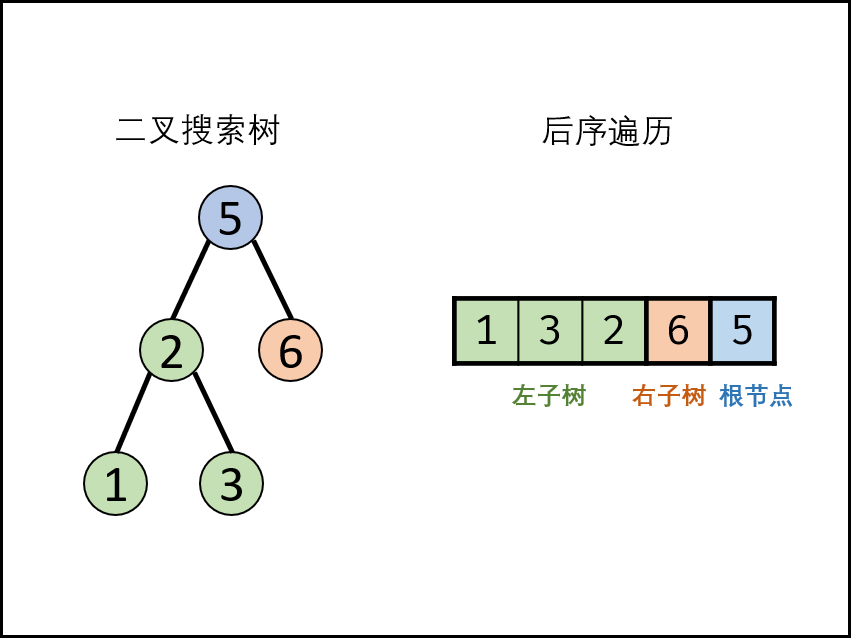##### 递归解析：
• 终止条件： 当 left ≥ right，说明此子树节点数量 ≤ 1 ，无需判别正确性，因此直接返回 true；

• 递推工作：

1. 划分左右子树： 遍历后序遍历的 [left,right] 区间元素，寻找 第一个大于根节点 的节点，索引记为 m 。

• 此时，可划分出左子树区间 [left,m−1]
• 右子树区间 [m,right−1]
• 根节点索引 right
1. 判断是否为二叉搜索树：

• 左子树区间 [left,m−1] 内的所有节点都应 < postorder[right]。
• 右子树区间 [m,right−1] 内的所有节点都应 > postorder[right] 。
• 返回值： 所有子树都需正确才可判定正确，因此使用 与逻辑符 && 连接。

1. p = right ： 判断 此树 是否正确。
2. recur(left,m−1) ： 判断 此树的左子树 是否正确。
3. recur(m,right−1)： 判断 此树的右子树 是否正确。
class Solution {
public boolean verifyPostorder(int[] postorder) {
return recur(postorder, 0, postorder.length - 1);
}
boolean recur(int[] postorder, int left, int right) {
if(left >= right) return true;
int rootValue = postorder[right]; // 根节点的值
int p = left;
while(postorder[p] < rootValue) p++; //左子树
int m = p;
while(postorder[p] > rootValue) p++; //右子树
return p == right && recur(postorder, left, m - 1) && recur(postorder, m, right - 1);
}
}


### 复杂度

• 时间复杂度 O(N2) ： 每次调用 recur(left,right) 减去一个根节点，因此递归占用 O(N) ；最差情况下（即当树退化为链表），每轮递归都需遍历树所有节点，占用 O(N) 。
• 空间复杂度 O(N) ： 最差情况下（即当树退化为链表），递归深度将达到 N 。

# 第 21 天 位运算（简单）

## 15. 二进制中1的个数

### 题目

输入：n = 11 (控制台输入 00000000000000000000000000001011)



输入：n = 128 (控制台输入 00000000000000000000000010000000)



输入：n = 4294967293 (控制台输入 11111111111111111111111111111101，部分语言中 n = -3）



### 解答

#### 方法1：调用API

public class Solution {
// you need to treat n as an unsigned value
public int hammingWeight(int n) {
return Integer.bitCount(n);
}
}


#### 方法2：逐位判断

• 根据 与运算 定义，设二进制数字 n ，则有：
• 若 n&1=0 ，则 n 二进制 最右一位 为 0 ；
• 若 n&1=1，则 n 二进制 最右一位 为 1 。
• 根据以上特点，考虑以下 循环判断
1. 判断 n 最右一位是否为 1 ，根据结果计数。
2. 将 n 右移一位（本题要求把数字 n 看作无符号数，因此使用 无符号右移(>>>) 操作）。
public class Solution {
public int hammingWeight(int n) {
int res = 0;
while(n != 0){
if((n&1) == 1)
res++;
n = n>>>1;
}
return res;
}
}

//优化
public class Solution {
public int hammingWeight(int n) {
int res = 0;
while(n != 0) {
res += n & 1;
n >>>= 1;
}
return res;
}
}


#### 方法3：巧用 n & (n - 1)

• (n−1) 解析： 二进制数字 n 最右边的 1 变成 0 ，此 1 右边的 0 都变成 1 。
• n&(n−1) 解析： 二进制数字 n 最右边的 1 变成 0 ，其余不变。即消去最右边 1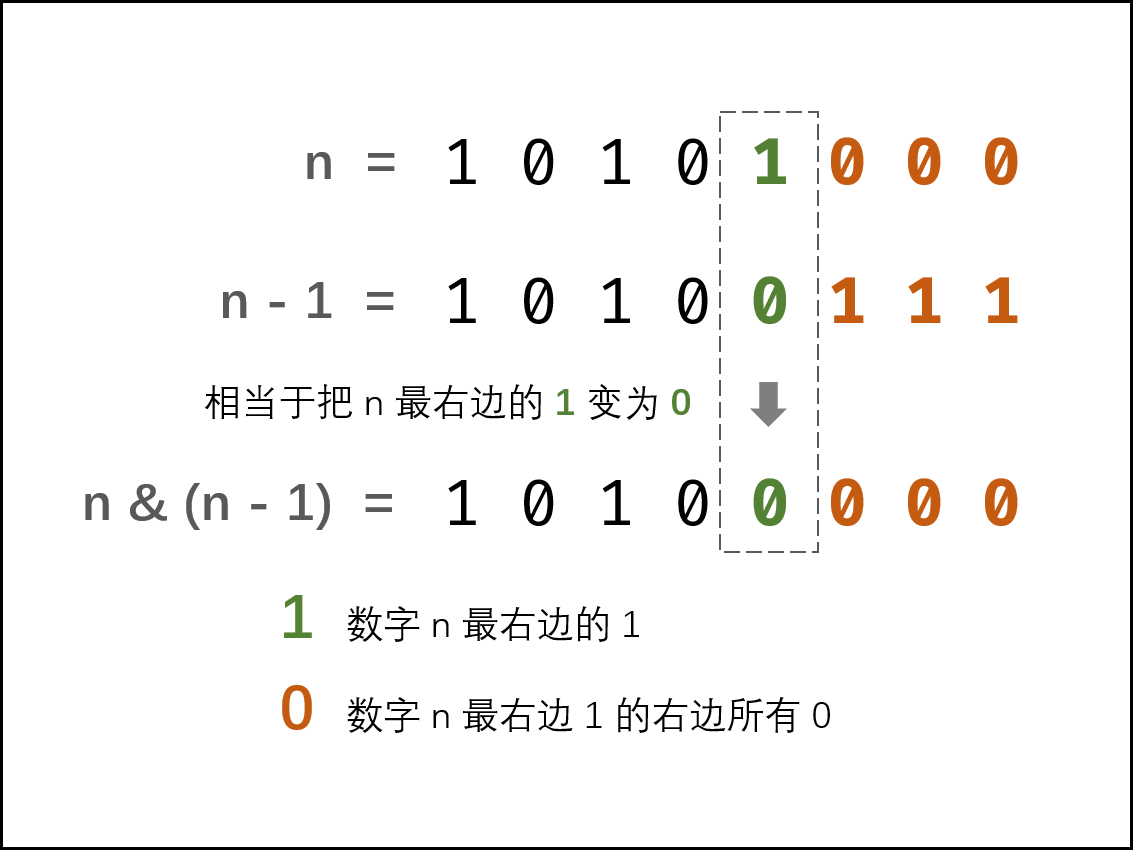public class Solution {
public int hammingWeight(int n) {
int res = 0;
while(n != 0) {
res++;
n &= n - 1;
}
return res;
}
}


### 复杂度

#### 方法2

• 时间复杂度 O(log2n)： 此算法循环内部仅有 移位、与、加 等基本运算，占用 O(1) ；逐位判断需循环 log2n 次，其中 log2n 代表数字 n 最高位 1 的所在位数（例如 log24=2, log216=4）。
• 空间复杂度 O(1) ： 变量 res 使用常数大小额外空间。

#### 方法3

• 时间复杂度 O(M) ： 循环内部仅有减法、加、与运算，占用 O(1) ；设 M 为二进制数字 n 中 1 的个数，则需循环 M 次（每轮消去一个 1 ），占用 O(M) 。
• 空间复杂度 O(1) ： 变量 res 使用常数大小额外空间。

## 65. 不用加减乘除做加法

### 题目

输入: a = 1, b = 1



### 解答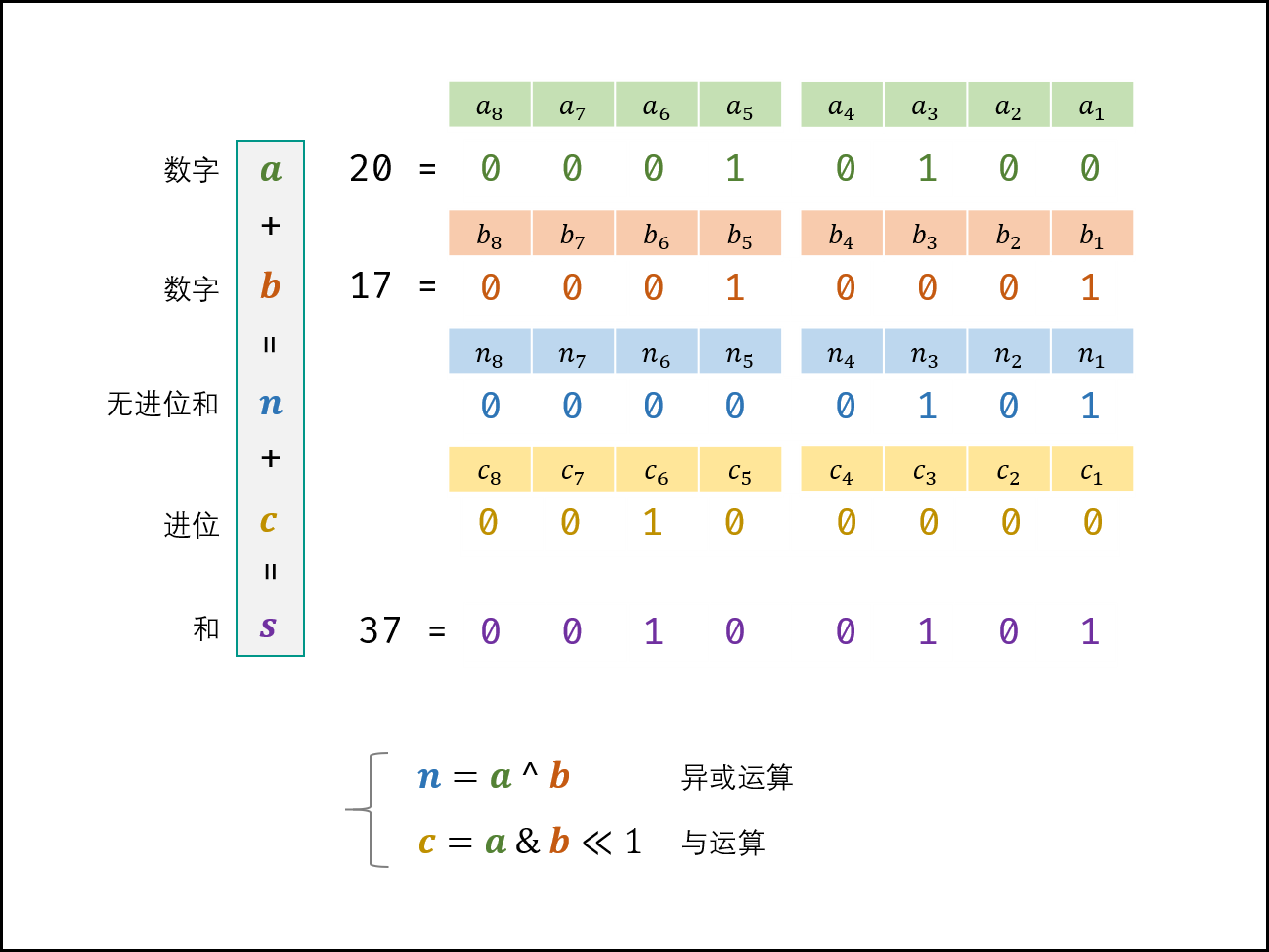class Solution {
public int add(int a, int b) {
while(b != 0) { // 当进位为 0 时跳出
int c = (a & b) << 1;  // c = 进位
int n = a ^ b; // n = 非进位和
a = n;  // a = 非进位和
b = c; // b = 进位
}
return a;
}
}

//优化
class Solution {
public int add(int a, int b) {
while(b != 0) { // 当进位为 0 时跳出
int c = (a & b) << 1;  // c = 进位
a ^= b; // a = 非进位和
b = c; // b = 进位
}
return a;
}
}


### 复杂度

• 时间复杂度 O(1) ： 最差情况下（例如 a= 0x7fffffff , b=1 时），需循环 32 次，使用 O(1) 时间；每轮中的常数次位操作使用 O(1) 时间。
• 空间复杂度 O(1) ： 使用常数大小的额外空间。

# 第 22 天 位运算（中等）

## 56 - I. 数组中数字出现的次数

### 解答

a⊕a⊕b⊕b⊕...⊕x
=0⊕0⊕...⊕x
=x

public int[] singleNumber(int[] nums) {
int x = 0;
for(int num : nums)  // 1. 遍历 nums 执行异或运算
x ^= num;
return x;            // 2. 返回出现一次的数字 x
}


a⊕a⊕b⊕b⊕...⊕x⊕y
=0⊕0⊕...⊕x⊕y
=x⊕y


• 若 (x⊕y) & 0001 = 1 ，则 x⊕y 的右边第一位为 1 ；
• 若 (x⊕y) & 0010 = 1，则 x⊕y 的右边第二位为 1 ；
• 以此类推……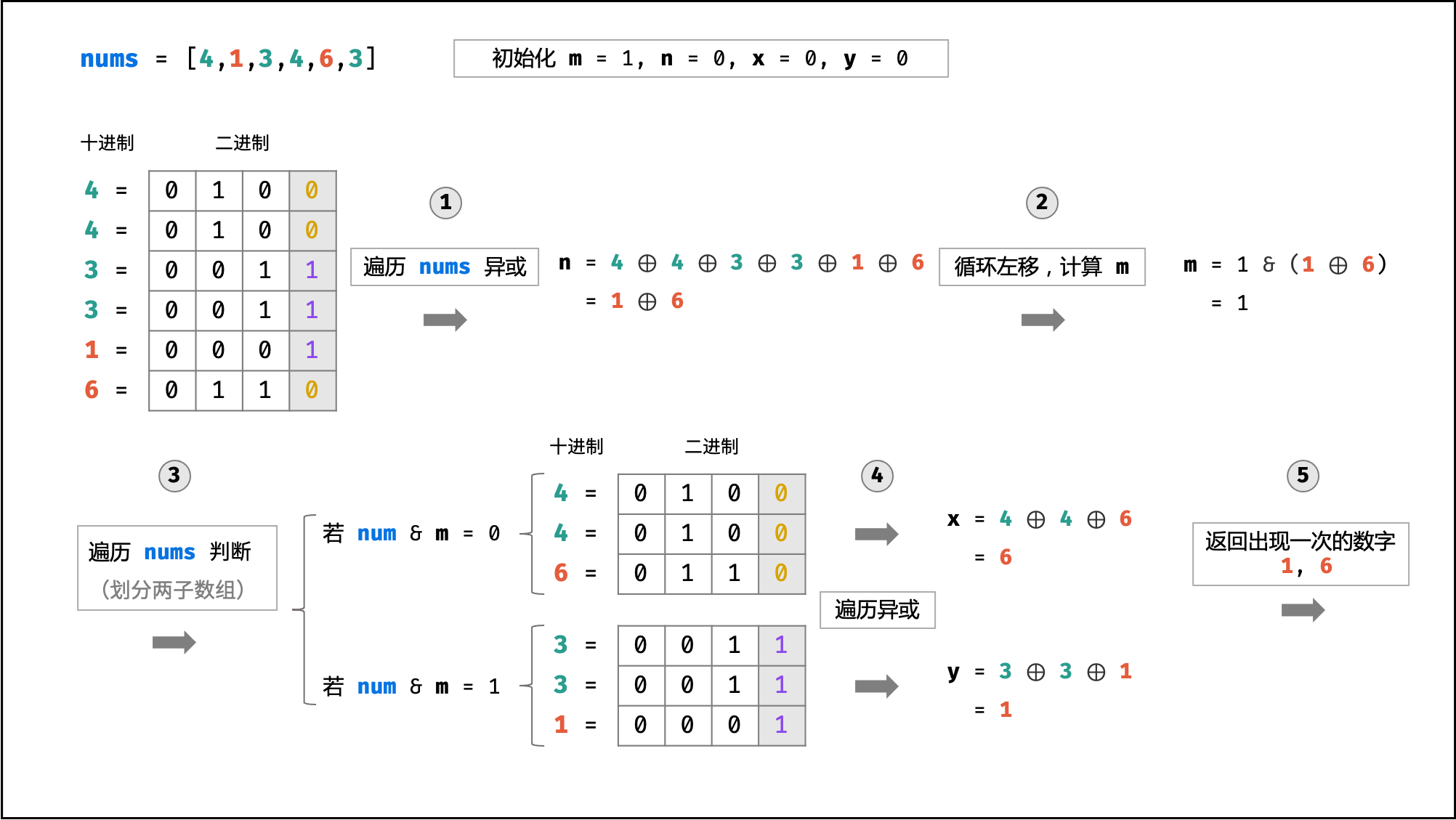class Solution {
public int[] singleNumbers(int[] nums) {
int n = 0, m = 1, x = 0, y = 0;
for(int num : nums)
n ^= num;
while((n & m) == 0)
m <<= 1;
for(int num : nums)
if((num & m) == 0)
x ^= num;
else y ^= num;
return new int[]{x,y};
}
}


### 复杂度

• 时间复杂度 O(N) ： 线性遍历 nums 使用 O(N) 时间，遍历 x⊕y 二进制位使用 O(32)=O(1) 时间。
• 空间复杂度 O(1) ： 辅助变量 n , m , x , y 使用常数大小额外空间。

## 56 - II. 数组中数字出现的次数 II

### 题目

输入：nums = [3,4,3,3]



输入：nums = [9,1,7,9,7,9,7]



### 解答

#### 方法1：哈希表

class Solution {
public int singleNumber(int[] nums) {
Map<Integer, Integer> map = new HashMap<>();
// 加入哈希表
for(int i = 0; i < nums.length; i++) {
map.put(nums[i], map.getOrDefault(nums[i], 0) + 1);
}
// 遍历找出第一个次数为1的数
for(int i = 0; i < nums.length; i++) {
if(map.get(nums[i]) == 1) {
return nums[i];
}
}
return -1;
}
}


#### 方法2：位运算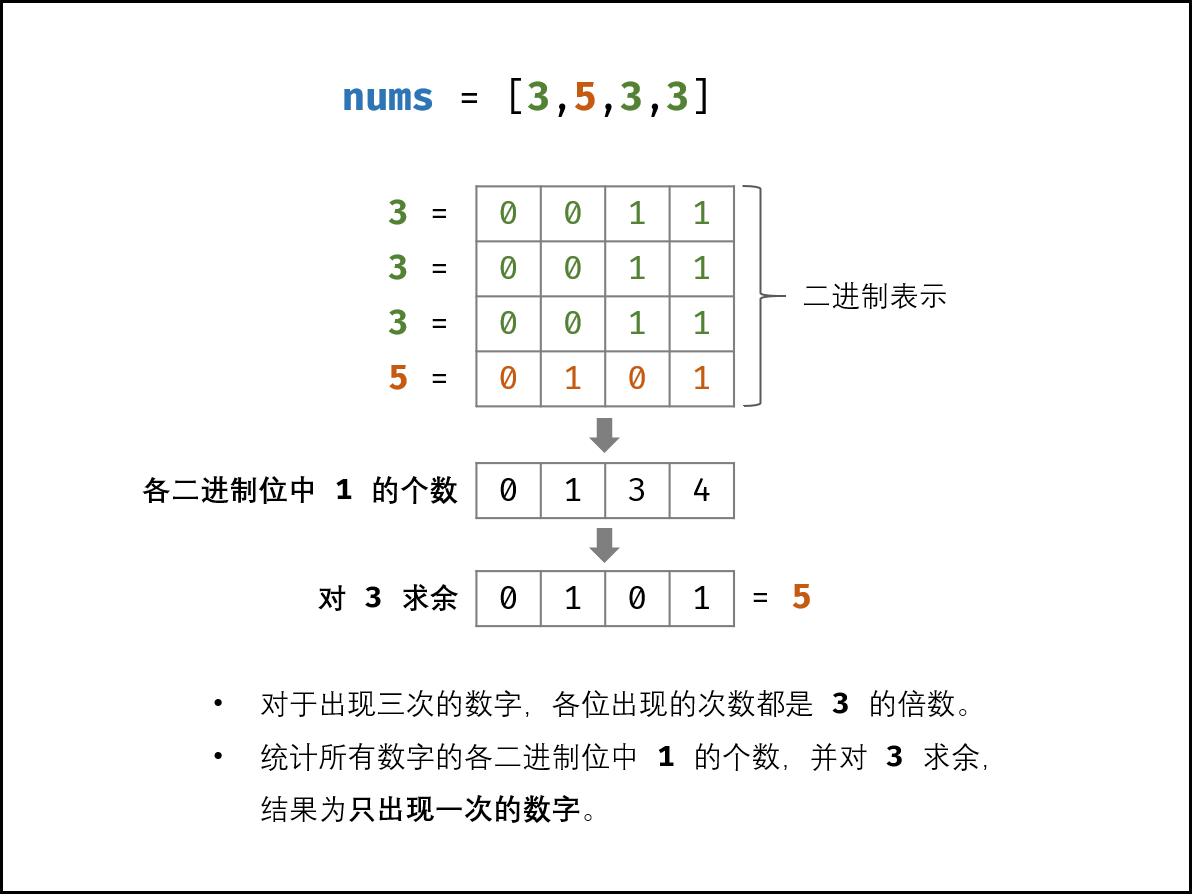n1=num&i

num=num>>>1

int[] counts = new int;
for(int i = 0; i < nums.length; i++) {
for(int j = 0; j < 32; j++) {
counts[j] += nums[i] & 1; // 更新第 j 位
nums[i] >>>= 1; // 第 j 位 --> 第 j + 1 位
}
}


for(int i = 0; i < 32; i++) {
counts[i] %= 3; // 得到 只出现一次的数字 的第 (31 - i) 位
}


for(int i = 0; i < counts.length; i++) {
res <<= 1; // 左移 1 位
res |= counts[31 - i]; // 恢复第 i 位的值到 res
}


class Solution {
public int singleNumber(int[] nums) {
int[] counts = new int;
for(int num : nums) {
for(int j = 0; j < 32; j++) {
counts[j] += num & 1;
num >>>= 1;
}
}
int res = 0, m = 3;
for(int i = 0; i < 32; i++) {
res <<= 1;
res |= counts[31 - i] % m;
}
return res;
}
}


### 复杂度

#### 方法1

• 时间复杂度 O(N) ： 其中 N 为数组 nums 的长度；遍历数组占用 O(N)
• 空间复杂度 O(N) ： HashMap 使用 O(N) 额外空间

#### 方法2

• 时间复杂度 O(N) ： 其中 NNN 位数组 nums 的长度；遍历数组占用 O(N) ，每轮中的常数个位运算操作占用 O(1) 。
• 空间复杂度 O(1) ： 数组 counts 长度恒为 32 ，占用常数大小的额外空间。

# 第 23 天 数学（简单）

## 39. 数组中出现次数超过一半的

### 题目

输入: [1, 2, 3, 2, 2, 2, 5, 4, 2]



### 解答

#### 方法1：哈希表

class Solution {
public int majorityElement(int[] nums) {
Map<Integer, Integer> map = new HashMap<>();
for(int i = 0; i < nums.length; i++){
map.put(nums[i], map.getOrDefault(nums[i], 0) + 1);
}
for(int i = 0; i < nums.length; i++){
if(map.get(nums[i]) > (nums.length/2)){
return nums[i];
}
}
return 0;
}
}

//优化
class Solution {
public int majorityElement(int[] nums) {
Map<Integer, Integer> map = new HashMap<>();
for(int num : nums){
map.put(num, map.getOrDefault(num, 0) + 1);
if(map.get(num) > nums.length/2)
return num;
}
return 0;
}
}


#### 方法2：排序

class Solution {
public int majorityElement(int[] nums) {
Arrays.sort(nums);
return nums[nums.length/2];
}
}


#### 方法3：摩尔投票法

• 推论一： 若记 众数 的票数为 +1 ，非众数 的票数为 −1 ，则一定有所有数字的票数和 > 0

• 推论二： 若数组的前 a 个数字的 票数和 =0 ，则 数组剩余 (n-a) 个数字的 票数和一定仍 >0 ，即后 (n-a) 个数字的众数仍为 x##### 算法流程:
1. 初始化： 票数统计 votes = 0 ， 众数 x = 0
2. 循环： 遍历数组 nums 中的每个数字 num
1. 当 票数 votes 等于 0 ，则假设当前数字 num 是众数；
2. num = x 时，票数 votes 自增 1 ；当 num != x 时，票数 votes 自减 1 ；
3. 返回值： 返回 x 即可；
class Solution {
public int majorityElement(int[] nums) {
int votes = 0, x = 0;
for(int num : nums){
if(votes == 0) x = num;
}
return x;
}
}

//优化
class Solution {
public int majorityElement(int[] nums) {
int x = 0, votes = 0;
for(int num : nums){
if(votes == 0) x = num;
votes += num == x ? 1 : -1;
}
return x;
}
}


### 复杂度

#### 方法1

• 时间复杂度 O(N) ： 其中 N 为数组 nums 的长度；遍历数组占用 O(N)
• 空间复杂度 O(N) ： HashMap 使用 O(N) 额外空间

#### 方法2

**时间复杂度 O(nlogn) **

### 方法3

• 时间复杂度 O(N)： N 为数组 nums 长度。
• 空间复杂度 O(1) ： votes 变量使用常数大小的额外空间。

## 66. 构建乘积数组

### 题目

输入: [1,2,3,4,5]



### 解答class Solution {
public int[] constructArr(int[] a) {
int len = a.length;
if(len == 0) return new int;
int[] b = new int[len];
b = 1;
int tmp = 1;
for(int i = 1; i < len; i++) {//计算下三角
b[i] = b[i - 1] * a[i - 1];
}
for(int i = len - 2; i >= 0; i--) {//计算上三角
tmp *= a[i + 1];
b[i] *= tmp;
}
return b;
}
}

class Solution {
public int[] constructArr(int[] a) {
int n = a.length;
int[] B = new int[n];
for (int i = 0, product = 1; i < n; product *= a[i], i++)       /* 从左往右累乘 */
B[i] = product;
for (int i = n - 1, product = 1; i >= 0; product *= a[i], i--)  /* 从右往左累乘 */
B[i] *= product;
return B;
}
}


### 复杂度

• 时间复杂度 O(N)： 其中 N 为数组长度，两轮遍历数组 a ，使用 O(N) 时间。
• 空间复杂度 O(1)： 变量 tmp 使用常数大小额外空间（数组 b 作为返回值，不计入复杂度考虑）。

# 第 24 天 数学（中等）

## 14- I. 剪绳子

### 题目

输入: 2



输入: 10



### 解答

• ① 当所有绳段长度相等时，乘积最大。

• ② 最优的绳段长度为 3 。

##### 切分规则：
1. 最优： 3 。把绳子尽可能切为多个长度为 3 的片段，留下的最后一段绳子的长度可能为 0,1,2 三种情况。
2. 次优： 2 。若最后一段绳子长度为 2 ；则保留，不再拆为 1+1 。
3. 最差： 1 。若最后一段绳子长度为 1 ；则应把一份 3+1 替换为 2+2，因为 2×2>3×1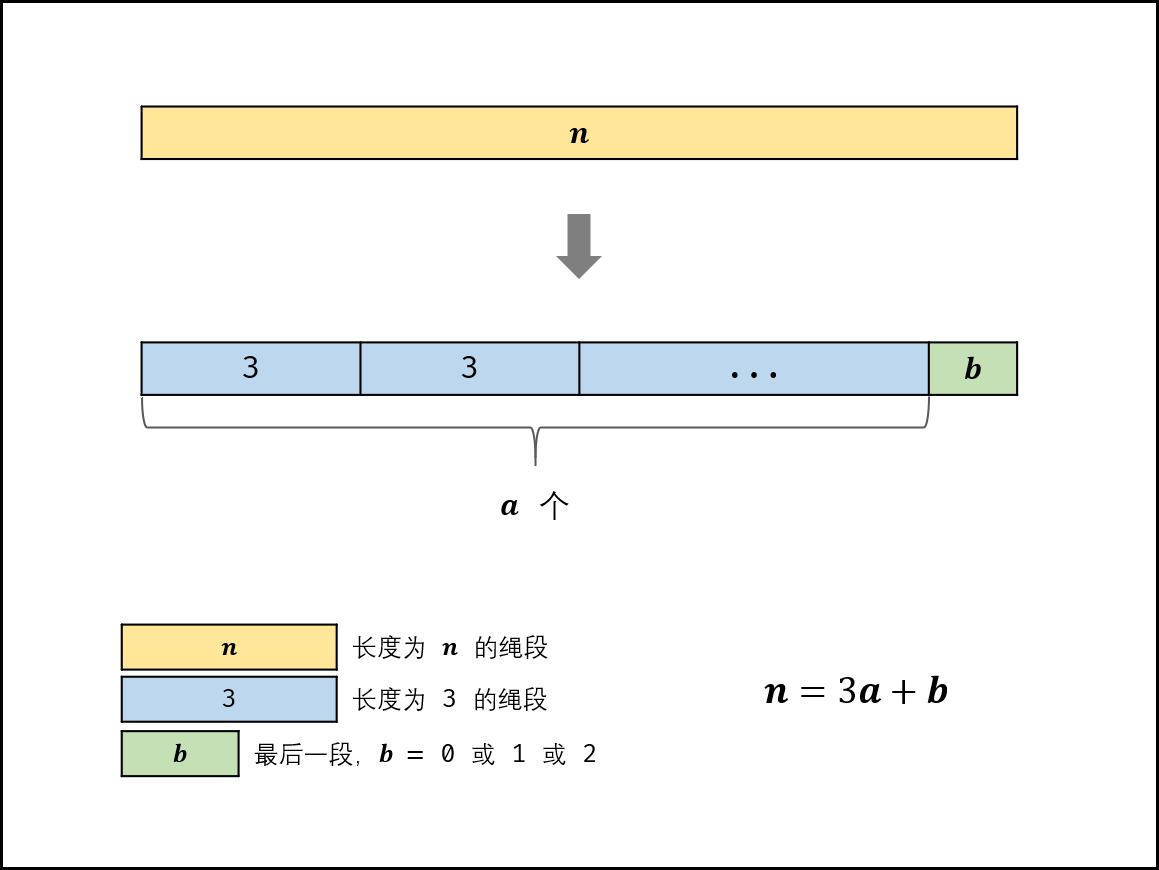##### 算法流程：
1. 当 n ≤ 3 时，按照规则应不切分，但由于题目要求必须剪成 m>1 段，因此必须剪出一段长度为 1 的绳子，即返回 n−1 。
2. 当 n > 3 时，求 n 除以 3 的 整数部分 a 和 余数部分 b （即 n=3a+b），并分为以下三种情况：
• 当 b = 0 时，直接返回 3a
• 当 b = 1 时，要将一个 1+3 转换为 2+2，因此返回 3a-1×4；
• 当 b=2 时，返回 3a×2。
class Solution {
public int cuttingRope(int n) {
if(n <= 3) return n - 1;
int a = n / 3;
int b = n % 3;
if(b == 0) return (int)Math.pow(3,a);
if(b == 1) return (int)Math.pow(3,a-1)*4;
return (int)Math.pow(3,a) * 2;
}
}


### 复杂度

• 时间复杂度 O(1) ： 仅有求整、求余、次方运算。
• 空间复杂度 O(1) ： 变量 ab 使用常数大小额外空间。

## 57 - II. 和为s的连续正数序列

### 题目

输入：target = 9



输入：target = 15



### 解答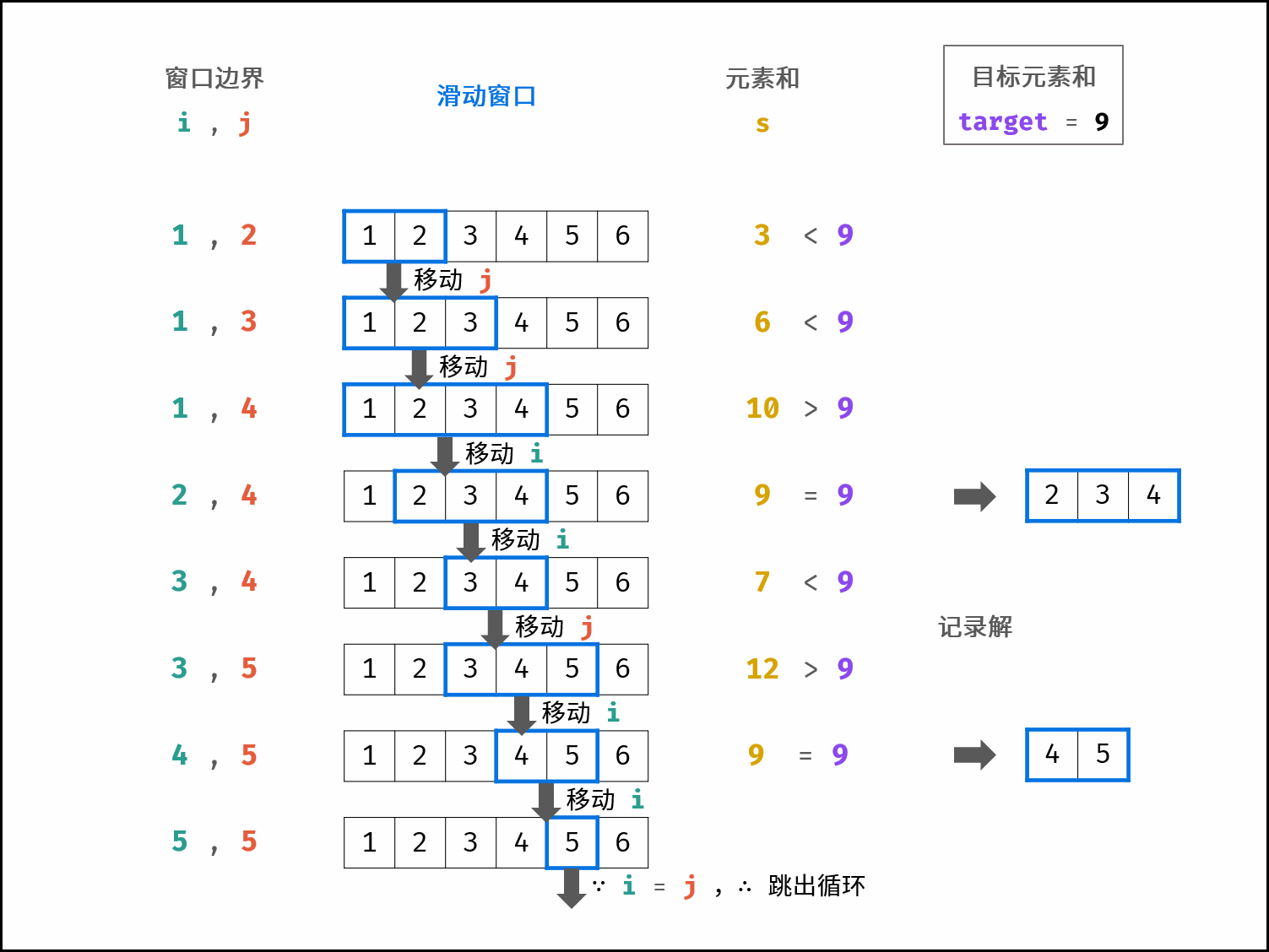##### 算法流程：
1. 初始化： 左边界 i=1，右边界 j=2，元素和 s=3 ，结果列表 res ；

2. 循环： 当 i ≥ j 时跳出；

• 当 s > target 时： 更新元素和 s，并向右移动左边界 i=i+1 ；
• 当 s < target 时： 向右移动右边界 j=j+1 ，并更新元素和 s ；
• 当 s = target 时： 记录连续整数序列，并向右移动左边界 i=i+1，更新元素和 s ；
3. 返回值： 返回结果列表 res ；

class Solution {
public int[][] findContinuousSequence(int target) {
int i = 1, j = 2, s = 3;
List<int[]> res = new ArrayList<>();
while(i < j){
if(s > target) {
s -= i;
i++;
}
else if(s < target){
j++;
s += j;
}
else{
int[] ans = new int[j-i+1];
for(int k = i; k <= j; k++)
ans[k - i] = k;
s -= i;
i++;

}
}
return res.toArray(new int[]);
}
}


### 复杂度

• 时间复杂度 O(N) ： 其中 N=target；连续整数序列至少有两个数字，而 i<j恒成立，因此至多循环 target 次；
• 空间复杂度 O(1) ： 变量 i , j , s 使用常数大小的额外空间。

## 62.圆圈中最后剩下的数字

### 题目

0,1,···,n-1这n个数字排成一个圆圈，从数字0开始，每次从这个圆圈里删除第m个数字（删除后从下一个数字开始计数）。求出这个圆圈里剩下的最后一个数字。

输入: n = 5, m = 3



输入: n = 10, m = 17



### 解答

f(n,m) 表示在 n 个数字(0～n-1)中不断删除第 m 个数字最后剩下的数字。删除一个数字后剩下了 n-1 个数字，那么再次删除就是在 n-1 个数字中删除第 m 个数字，可以表示为 f(n-1,m)

f'(n-1,m)表示序列: k+1 k+2 k+3 ... n-1     0     1  ... k-1
f(n-1,m) 表示序列:  0   1   2  ... n-k-2 n-k-1  n-k ... n-2


因为
f'(n-1,m)=(f(n-1,m)+k+1)%n
k+1= m%n
f(n,m) = f'(n-1,m)

f(n-1,m)=[f(n-1,m)+m]%n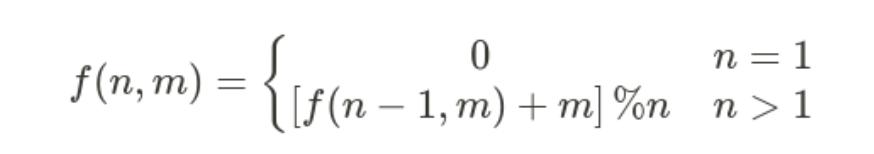##### 动态规划解析：
1. 状态定义： 设「i,m问题」的解为 dp[i]；

2. 转移方程： 通过以下公式可从 dp[i−1] 递推得到 dp[i] = (dp[i−1] + m) % i；

3. 初始状态：「1,m 问题」的解恒为 0 ，即 dp = 0；

4. 返回值： 返回「n,m 问题」的解 dp[n] ；

class Solution {
public int lastRemaining(int n, int m) {
int dp[] = new int[n+1];
dp = 0;
for(int i = 2; i <= n; i++){
dp[i] = (dp[i-1] + m) % i;
}
return dp[n];
}
}

//优化
//根据状态转移方程的递推特性，无需建立状态列表 dp ，而使用一个变量 x 执行状态转移即可。
class Solution {
public int lastRemaining(int n, int m) {
int x = 0;
for (int i = 2; i <= n; i++) {
x = (x + m) % i;
}
return x;
}
}


### 复杂度

• 时间复杂度 O(n)： 状态转移循环 n−1次使用 O(n)时间，状态转移方程计算使用 O(1) 时间；
• 空间复杂度 O(1)： 使用常数大小的额外空间；

# 第 25 天 模拟（中等）

## 29. 顺时针打印矩阵

### 题目

输入：matrix = [[1,2,3],[4,5,6],[7,8,9]]



输入：matrix = [[1,2,3,4],[5,6,7,8],[9,10,11,12]]



### 解答

1. 空值处理：matrix 为空时，直接返回空列表 [] 即可。
2. 初始化： 矩阵 左、右、上、下 四个边界 left , right , top , bottom ，用于打印的结果列表 res
3. 循环打印： “从左向右、从上向下、从右向左、从下向上” 四个方向循环，每个方向打印中做以下三件事 （各方向的具体信息见下表）
1. 根据边界打印，即将元素按顺序添加至列表 res 尾部；
2. 边界向内收缩 1 （代表已被打印）；
3. 判断是否打印完毕（边界是否相遇），若打印完毕则跳出。
4. 返回值： 返回 res 即可。

class Solution {
public int[] spiralOrder(int[][] matrix) {
if(matrix.length == 0) return new int;
int left = 0, right = matrix.length -1, top = 0, bottom = matrix.length -1, count = 0;
int[] res = new int[(right + 1)*(bottom + 1)];
while(true){
for(int i = left; i <= right; i++){ // 从左到右
res[count++] = matrix[top][i];
}
if(++top > bottom) break;
for(int i = top; i <= bottom; i++){ // 从上到下
res[count++] = matrix[i][right];
}
if(left > --right) break;
for(int i = right; i >= left; i--){ // 从右到左
res[count++] = matrix[bottom][i];
}
if(top > --bottom) break;
for(int i = bottom; i >= top; i--){ // 从下到上
res[count++] = matrix[i][left];
}
if(++left > right) break;
}
return res;
}
}


### 复杂度

• 时间复杂度 O(MN)： M,N 分别为矩阵行数和列数。
• 空间复杂度 O(1) ： 四个边界使用常数大小的 额外 空间（ res 为必须使用的空间）。

## 31. 栈的压入、弹出序列

### 题目

输入：pushed = [1,2,3,4,5], popped = [4,5,3,2,1]

push(1), push(2), push(3), push(4), pop() -> 4,
push(5), pop() -> 5, pop() -> 3, pop() -> 2, pop() -> 1


输入：pushed = [1,2,3,4,5], popped = [4,3,5,1,2]



### 解答

• 入栈操作： 按照压栈序列的顺序执行。
• 出栈操作： 每次入栈后，循环判断 “栈顶元素 === 弹出序列的当前元素” 是否成立，将符合弹出序列顺序的栈顶元素全部弹出。

1. 初始化： 辅助栈 stack，弹出序列的索引 i ；
2. 遍历压栈序列： 各元素记为 num ；
1. 元素 num 入栈；
2. 循环出栈：若 stack 的栈顶元素 === 弹出序列元素 popped[i] ，则执行出栈与 i++ ；
3. 返回值： 若 stack 为空，则此弹出序列合法。
class Solution {
public boolean validateStackSequences(int[] pushed, int[] popped) {
Stack<Integer> stack = new Stack<>();
int i = 0;
for(int num : pushed) {
stack.push(num); // num 入栈
while(!stack.isEmpty() && stack.peek() == popped[i]) { // 循环判断与出栈
stack.pop();
i++;
}
}
return stack.isEmpty();
}
}


### 复杂度

• 时间复杂度 O(N) ： 其中 N 为列表 pushed 的长度；每个元素最多入栈与出栈一次，即最多共 2N 次出入栈操作。
• 空间复杂度 O(N) ： 辅助栈 stack 最多同时存储 N 个元素。

# 第 26 天 字符串（中等）

## 20. 表示数值的字符串

### 题目

1. 若干空格
2. 一个 小数 或者 整数
3. （可选）一个 'e''E' ，后面跟着一个 整数
4. 若干空格

1. （可选）一个符号字符（'+''-'
2. 下述格式之一：
3. 至少一位数字，后面跟着一个点 '.'
4. 至少一位数字，后面跟着一个点 '.' ，后面再跟着至少一位数字
5. 一个点 '.' ，后面跟着至少一位数字

1. （可选）一个符号字符（'+''-'
2. 至少一位数字

• ["+100", "5e2", "-123", "3.1416", "-1E-16", "0123"]

• ["12e", "1a3.14", "1.2.3", "+-5", "12e+5.4"]

输入：s = "0"



输入：s = "e"



输入：s = "."



输入：s = "    .1  "



### 解答

#### 方法1：暴力

class Solution {
public boolean isNumber(String s) {
if (s == null || s.length() == 0) return false;// s为空对象或s长度为0,不表示数值
//是否出现数字、小数点、e或者E
boolean numFlag = false, dotFlag = false, eFlag = false;
//去掉首尾空格
s = s.trim();
for (int i = 0; i < s.length(); i++) {
//判定为数字，则标记numFlag
if (s.charAt(i) >= '0' && s.charAt(i) <= '9') {
numFlag = true;
//小数点只可以出现再e之前，且只能出现一次.num  num.num num.都是被允许的
} else if (s.charAt(i) == '.' && !dotFlag && !eFlag) {
dotFlag = true;
//判定为e，需要没出现过e，并且出过数字了
} else if ((s.charAt(i) == 'e' || s.charAt(i) == 'E') && !eFlag && numFlag) {
eFlag = true;
numFlag = false;//重置，避免e以后没有出现数字，防止出现123e或者123e+的非法情况
//判定为+-符号，只能出现在第一位或者紧接e后面
} else if (s.charAt(i) == '+' || s.charAt(i) == '-') {
if(i != 0 && s.charAt(i - 1) != 'e' && s.charAt(i - 1) != 'E')
return false;
} else return false;//其他情况，都是非法的
}
//是否出现了数字
return numFlag;
}
}


• 时间复杂度：O(n)
• 空间复杂度：O(1)

## 67. 把字符串转换成整数

### 题目

输入: "42"



输入: "   -42"

我们尽可能将负号与后面所有连续出现的数字组合起来，最后得到 -42 。


输入: "4193 with words"



输入: "words and 987"



输入: "-91283472332"



### 解答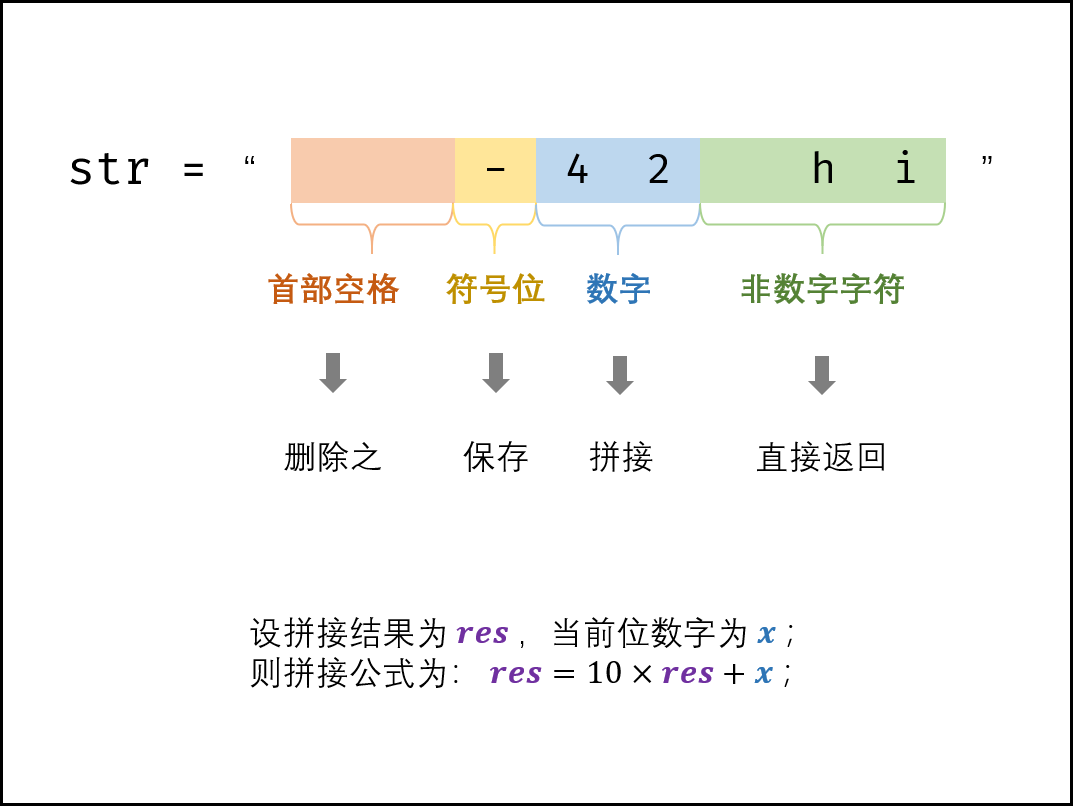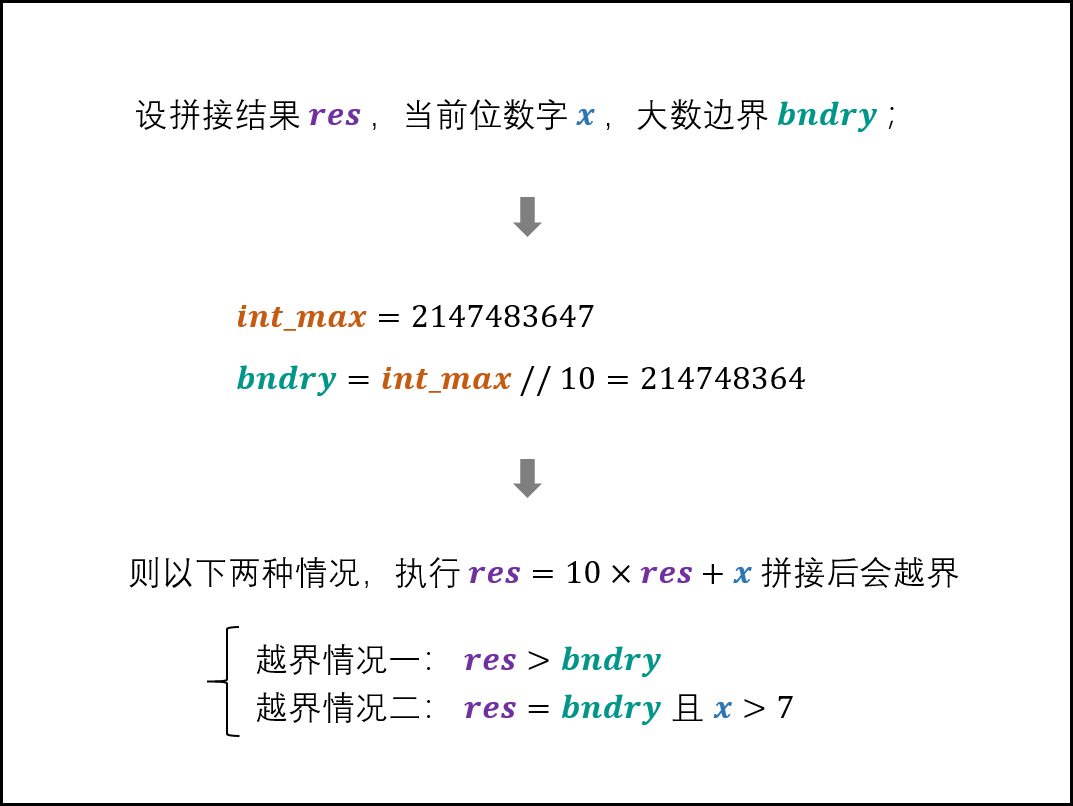• res > bndry 意思是，此时 res 已经大于 bndry 了，* 10 一定越界
• res == bndry && chars[j] > '7' 的意思是，当 res == bndry 时，即：214748364 此时 res * 10 变成 2147483640 此时没越界，但是还需要 + chars[j]，而 int 最大值为 2147483647，所以当chars[j] > 7 时会越界
class Solution {
public int strToInt(String str) {
//去前后空格
char[] chars = str.trim().toCharArray();
if (chars.length == 0) return 0;
//记录第一个符合是否为负数
int sign = 1;
//开始遍历的位置
int i = 1;
//如果首个非空格字符为负号，那么从位置1开始遍历字符串，并且结果需要变成负数
if (chars == '-') {
sign = -1;
} else if (chars != '+') { //如果首个非空格字符不是负号也不是加号，从第一个元素开始遍历
i = 0;
}
int bndry = Integer.MAX_VALUE / 10; // 214748364
int res = 0;
for (int j = i; j < chars.length; j++) {
//遇到非数字直接退出
if (chars[j] > '9' || chars[j] < '0') break;
//判断是否越界 2^31−1 = 2147483647
if (res > bndry || (res == bndry && chars[j] > '7')) {
//根据字符串首负号判断返回最大值还是最小值
return sign == 1 ? Integer.MAX_VALUE : Integer.MIN_VALUE;
}
//字符转数字：“此数字的ASCII码”与“0的ASCII码”相减即可
res = res * 10 + (chars[j] - '0');
}
//返回结果，需要判断正负
return res * sign;
}
}


### 复杂度

• 时间复杂度 O(N) ： 其中 N 为字符串长度，线性遍历字符串占用 O(N) 时间。
• 空间复杂度 O(N) ： 删除首尾空格后需建立新字符串，最差情况下占用 O(N) 额外空间。

# 第 27 天 栈与队列（困难）

## 59 - I. 滑动窗口的最大值

### 题目

输入: nums = [1,3,-1,-3,5,3,6,7], 和 k = 3

滑动窗口的位置                最大值
---------------               -----
[1  3  -1] -3  5  3  6  7       3
1 [3  -1  -3] 5  3  6  7       3
1  3 [-1  -3  5] 3  6  7       5
1  3  -1 [-3  5  3] 6  7       5
1  3  -1  -3 [5  3  6] 7       6
1  3  -1  -3  5 [3  6  7]      7


### 解答

1. deque 内 仅包含窗口内的元素 ⇒ 每轮窗口滑动移除了元素 nums[i−1]，需将 deque 内的对应元素一起删除。
2. deque 内的元素 非严格递减 ⇒ 每轮窗口滑动添加了元素 nums[j+1] ，需将 deque 内所有 <nums[j+1]的元素删除。
##### 算法流程：
1. 初始化： 双端队列 deque，结果列表 res ，数组长度 n ；
2. 滑动窗口： 左边界范围 i∈[1−k,n−k]，右边界范围 j∈[0,n−1] ；
1. 若 i>0 且 队首元素 deque = 被删除元素 nums[i−1] ，则队首元素出队；
2. 删除 deque 内所有 <nums[j] 的元素，以保持 deque 递减；
3. 将 nums[j] 添加至 deque 尾部；
4. 若已形成窗口（即 i≥0 ）：将窗口最大值（即队首元素 deque）添加至列表 res ；
3. 返回值： 返回结果列表 res ；
class Solution {
public int[] maxSlidingWindow(int[] nums, int k) {
if(nums.length == 0 || k == 0) return new int;
int[] res = new int[nums.length - k + 1];
for(int j = 0, i = 1 - k; j < nums.length; i++, j++) {
// 删除 deque 中对应的 nums[i-1]
if(i > 0 && deque.peekFirst() == nums[i - 1])
deque.removeFirst();
// 保持 deque 递减
while(!deque.isEmpty() && deque.peekLast() < nums[j])
deque.removeLast();
// 记录窗口最大值
if(i >= 0)
res[i] = deque.peekFirst();
}
return res;
}
}


### 复杂度

• 时间复杂度 O(n) ： 其中 n 为数组 nums 长度；线性遍历 nums 占用 O(n) ；每个元素最多仅入队和出队一次，因此单调队列 deque 占用 O(2n)。
• 空间复杂度 O(k)： 双端队列 deque 中最多同时存储 k 个元素（即窗口大小）。

## 59 - II. 队列的最大值

### 题目

输入:
["MaxQueue","push_back","push_back","max_value","pop_front","max_value"]
[[],,,[],[],[]]



输入:
["MaxQueue","pop_front","max_value"]
[[],[],[]]



### 解答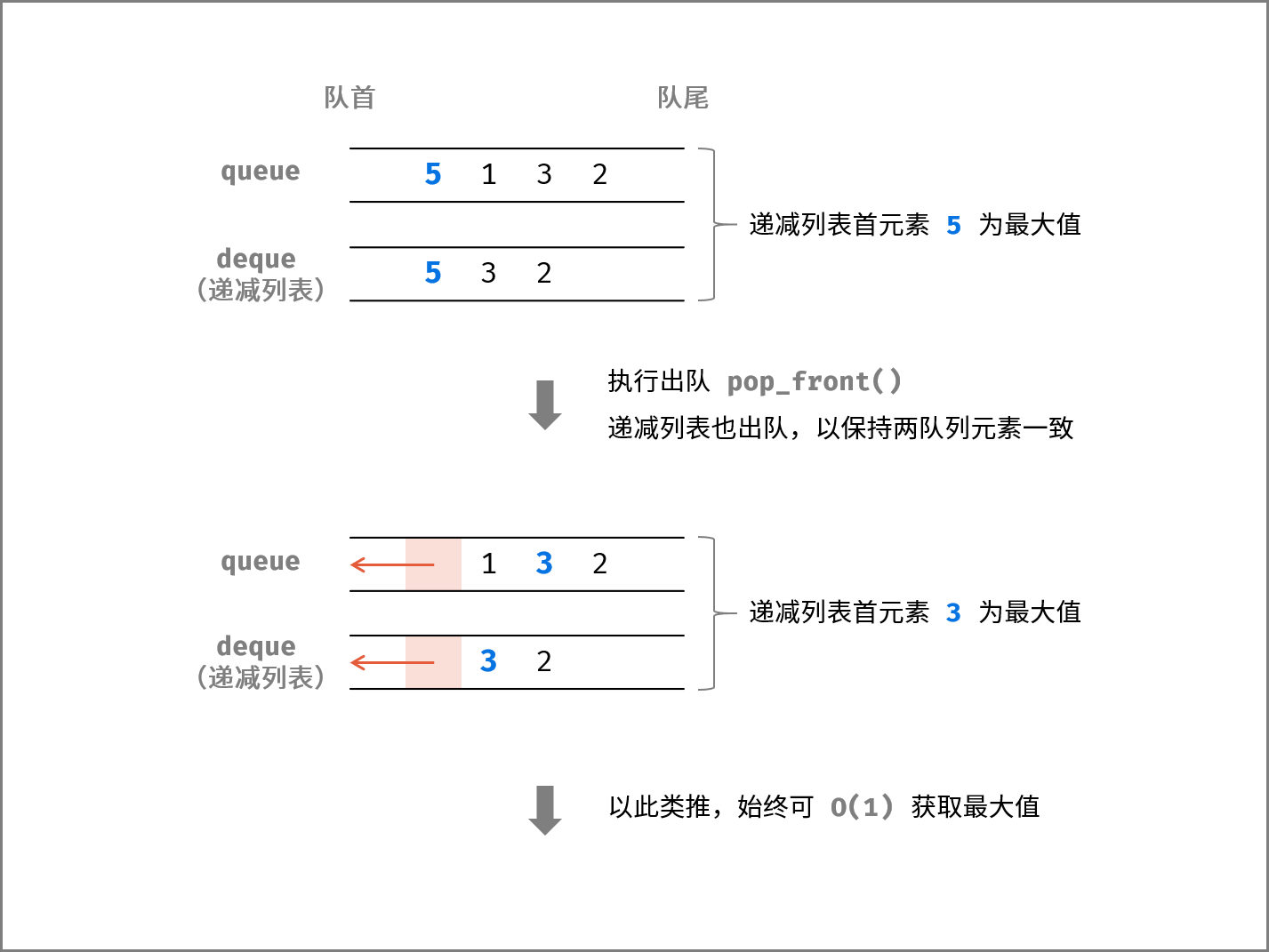1. 当执行入队 push_back() 时： 若入队一个比队列某些元素更大的数字 x ，则为了保持此列表递减，需要将双向队列 尾部所有小于 x 的元素 弹出。
2. 当执行出队 pop_front() 时： 若出队的元素是最大元素，则 双向队列 需要同时 将首元素出队 ，以保持队列和双向队列的元素一致性。
##### 函数设计：

• 当双向队列 deque 为空，则返回 −1 ；
• 否则，返回 deque 首元素；

1. 将元素 value 入队 queue
2. 将双向队列中队尾 所有 小于 value 的元素弹出（以保持 deque 非单调递减），并将元素 value 入队 deque

1. 若队列 queue 为空，则直接返回 −1 ；
2. 否则，将 queue 首元素出队；
3. deque 首元素和 queue 首元素 相等 ，则将 deque 首元素出队（以保持两队列 元素一致 ） ；
class MaxQueue {
Queue<Integer> queue;
Deque<Integer> deque;
public MaxQueue() {
}

public int max_value() {
return deque.isEmpty() ? -1 : deque.peekFirst();
}
//入队
public void push_back(int value) {
while(!deque.isEmpty() && deque.peekLast() < value)
deque.removeLast(); // deque.pollLast();
}
//出队
public int pop_front() {
if(queue.isEmpty()) return -1;
// queue 里面保存的是 Integer 而非 int ，peek() 返回的是 Integer 类型，没有自动拆箱，因此需要用 equals() 来比~
if(queue.peek().equals(deque.peekFirst()))
deque.removeFirst(); // deque.pollFirst();
return queue.remove(); // queue.poll();
}
}

/**
* Your MaxQueue object will be instantiated and called as such:
* MaxQueue obj = new MaxQueue();
* int param_1 = obj.max_value();
* obj.push_back(value);
* int param_3 = obj.pop_front();
*/


### 复杂度

• 时间复杂度 O(1) ： max_value(), push_back(), pop_front() 方法的均摊时间复杂度均为 O(1) ；
• 空间复杂度 O(N) ： 当元素个数为 N 时，最差情况下deque 中保存 N 个元素，使用 O(N) 的额外空间；

# 第 28 天 搜索与回溯算法（困难）

## 37. 序列化二叉树

### 题目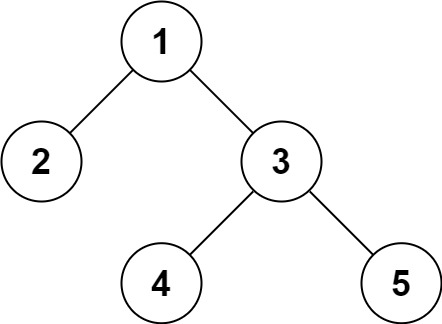输入：root = [1,2,3,null,null,4,5]



### 解答

#### 方法1：DFS

• 序列化
1. 递归的第一步都是特例的处理，因为这是递归的中止条件：如果根节点为空，返回”null“
2. 序列化的结果为：根节点值 + “,” + 左子节点值(进入递归) + “,” + 右子节点值(进入递归)
3. 递归就是不断将“根节点”值加到结果中的过程
• 反序列化
1. 先将字符串转换成队列
2. 接下来就进入了递归
i. 弹出左侧元素，即队列出队
ii. 如果元素为“null”，返回null
iii. 否则，新建一个节点，其值为弹出元素
iv. 其左子节点为队列的下一个元素，进入递归；右子节点为队列的下下个元素，也进入递归
v. 递归就是不断将子树的根节点连接到父节点的过程
public class Codec {

// Encodes a tree to a single string.
public String serialize(TreeNode root) {
if(root == null) return "null,";
String res = root.val + ",";
res += serialize(root.left);
res += serialize(root.right);
return res;
}

// Decodes your encoded data to tree.
public TreeNode deserialize(String data) {
String[] arr = data.split(",");
for(int i = 0; i < arr.length; i++){
queue.offer(arr[i]);
}
return dfs(queue);
}
public TreeNode dfs(Queue<String> queue){
String val = queue.poll();
if(val.equals("null")) return null;
TreeNode root = new TreeNode(Integer.valueOf(val));
root.left = dfs(queue);
root.right = dfs(queue);
return root;
}
}

//优化
public class Codec {

// Encodes a tree to a single string.
public String serialize(TreeNode root) {
if(root == null) return "null";
return root.val + "," + serialize(root.left) + "," + serialize(root.right);
}

// Decodes your encoded data to tree.
public TreeNode deserialize(String data) {
return dfs(queue);
}

private TreeNode dfs(Queue<String> queue) {
String val = queue.poll();
if("null".equals(val)) return null;
TreeNode root = new TreeNode(Integer.parseInt(val));
root.left = dfs(queue);
root.right = dfs(queue);
return root;
}
}


#### 方法2：BFS

• 序列化
1. 用BFS遍历树，与一般遍历的不同点是不管node的左右子节点是否存在，统统加到队列中
2. 在节点出队时，如果节点不存在，在返回值res中加入一个”null”；如果节点存在，则加入节点值的字符串形式
• 反序列化
1. 同样使用BFS方法，利用队列新建二叉树
2. 首先要将data转换成列表，然后遍历，只要不为null将节点按顺序加入二叉树中；同时还要将节点入队
3. 队列为空时遍历完毕，返回根节点
public class Codec {

// Encodes a tree to a single string.
public String serialize(TreeNode root) {
if(root == null){
return "[]";
}
StringBuilder res = new StringBuilder();
res.append("[");
queue.offer(root);
while(!queue.isEmpty()){
TreeNode node = queue.poll();
if (node != null){
res.append(node.val);
queue.offer(node.left);
queue.offer(node.right);
}else {
res.append("null");
}
res.append(",");
}
res.append("]");
return res.toString();
}

// Decodes your encoded data to tree.
public TreeNode deserialize(String data) {
if(data == "[]"){
return null;
}
String[] dataList = data.substring(1, data.length() - 1).split(",");
TreeNode root = new TreeNode(Integer.parseInt(dataList));
queue.offer(root);
int i = 1;
while(!queue.isEmpty()){
TreeNode node = queue.poll();
if(!dataList[i].equals("null")){
node.left = new TreeNode(Integer.parseInt(dataList[i]));
queue.offer(node.left);
}
i++;
if(!dataList[i].equals("null")){
node.right = new TreeNode(Integer.parseInt(dataList[i]));
queue.offer(node.right);
}
i++;
}
return root;
}
}


• 时间复杂度：O(n)
• 空间复杂度：O(n)

• 时间复杂度：O(n)
• 空间复杂度：O(n)

## 38. 字符串的排列

### 题目

输入：s = "abc"



### 解答




# 第 29 天 动态规划（困难）

## 19. 正则表达式匹配

### 题目

输入:
s = "aa"
p = "a"



输入:
s = "aa"
p = "a*"



输入:
s = "ab"
p = ".*"



输入:
s = "aab"
p = "c*a*b"



输入:
s = "mississippi"
p = "mis*is*p*."


• s 可能为空，且只包含从 a-z 的小写字母。
• p 可能为空，且只包含从 a-z 的小写字母以及字符 .*，无连续的 '*'

### 解答




## 49. 丑数

### 题目

输入: n = 10



### 解答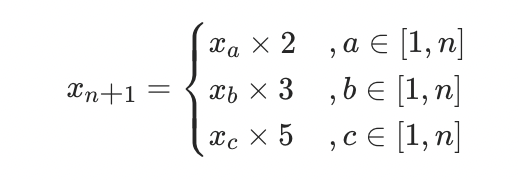##### 动态规划解析：
• 状态定义： 设动态规划列表 dp ，dp[i] 代表第 i+1 个丑数；

• 转移方程：

1. 当索引 a,b,c 满足以下条件时， dp[i] 为三种情况的最小值；
2. 每轮计算 dp[i] = min⁡(dp[a]×2, dp[b]×3, dp[c]×5) 后，需要更新索引 a,b,c 的值。实现方法：分别独立判断 dp[i] 和 dp[a]×2 , dp[b]×3 , dp[c]×5 的大小关系，若相等则将对应索引 a , b , c 加 1 ；
• 初始状态： dp=1 ，即第一个丑数为 1 ；

• 返回值： dp[n−1]，即返回第 n 个丑数；

class Solution {
public int nthUglyNumber(int n){
int a = 0, b = 0, c = 0;
int[] dp = new int[n];
dp = 1;
for(int i = 1; i < n; i++){
int x = dp[a] * 2, y = dp[b] * 3, z = dp[c] * 5;
dp[i] = Math.min(Math.min(x,y),z);
if(dp[i] == x) a++;
if(dp[i] == y) b++;
if(dp[i] == z) c++;
}
return dp[n-1];
}
}


### 复杂度

• 时间复杂度 O(N) ： 其中 N=n ，动态规划需遍历计算 dp 列表。
• 空间复杂度 O(N) ： 长度为 N 的 dp 列表使用 O(N) 的额外空间。

## 60. n个骰子的点数

### 题目

输入: 1



输入: 2



### 解答f(n−1,x−i)中的 x−i 会有越界问题。例如，若希望递推计算 f(2,2)，由于一个骰子的点数和范围为 [1,6] ，因此只应求和 f(1,1) ，即 f(1,0), f(1,−1), ..., f(1,−4) 皆无意义。因此需要 x−i > 0

// 自底向上的迭代解法
class Solution {
public double[] dicesProbability(int n) {
// n 个骰子可能扔出的结果的最大值和最小值
int min = n, max = n * 6;
// 定义：用 n 个骰子，凑出 x 的点数的概率是 dp[n][x]
double[][] dp = new double[n + 1][max + 1];
// 一个骰子扔出点数 1~6 的概率是 1/6
for (int j = 1; j <= 6; j++) {
dp[j] = 1 / 6.0;
}
// 状态转移
for (int i = 2; i <= n; i++) {
for (int j = i * 1; j <= i * 6; j++) {
for (int k = 1; k <= 6; k++) {
if (j - k <= 0) {
break;
}
// i 个骰子扔出点数 j 的概率
// 可以通过 i - 1 个骰子扔出点数 j - k 的概率推倒出来
dp[i][j] += dp[i - 1][j - k] * 1 / 6.0;
}
}
}
//点数和的范围为 [n, 6n] ，长度为 6n - n + 1 = 5n + 1
double[] res = new double[max - min + 1];
for (int i = 0; i < res.length; i++) {
res[i] = dp[n][min + i];
}
return res;
}
}


### 复杂度

• 时间复杂度 O(n2) ： 状态转移循环 n−1 轮；每轮中，当 i = 2,3,…,n 时，对应循环数量分别为 6×6,11×6,…,(5n+1)×6 ；因此总体复杂度为 O((n−1)×{[6+(5n+1)]/2}×6)，即等价于O(n2) 。
• 空间复杂度 O(n2)：n*(5n+1)

# 第 30 天 分治算法（困难）

## 17. 打印从1到最大的n位数

### 题目

输入: n = 1



### 解答

class Solution {
public int[] printNumbers(int n) {
int end = (int)Math.pow(10,n) - 1;
int[] res = new int[end];
for(int i = 0; i < end; i++){
res[i] = i + 1;
}
return res;
}
}


。。。。

### 复杂度

• 时间复杂度 O(10n) ： 生成长度为 10n 的列表需使用 O(10n) 时间。
• 空间复杂度 O(1) ： 建立列表需使用 O(1) 大小的额外空间（ 列表作为返回结果，不计入额外空间 ）。

## 51. 数组中的逆序对

### 解答




# 第 31 天 数学（困难）

## 14- II. 剪绳子 II

### 解答




## 43. 1～n 整数中 1 出现的次数

### 解答




## 44. 数字序列中某一位的数字

### 解答


`

### 复杂度

 支付宝支付 微信支付上一篇SpringBoot-基础篇

2022-09-06Redis-实战篇
⓪系统简介 短信登录：使用Redis共享session来实现 商户查询缓存：理解缓存击穿，缓存穿透，缓存雪崩等问题 优惠卷秒杀 学会Redis的计数器功能， 结合Lua完成高性能的redis操作 学会Redis分布式锁的原理，包括R
2022-08-08
目录